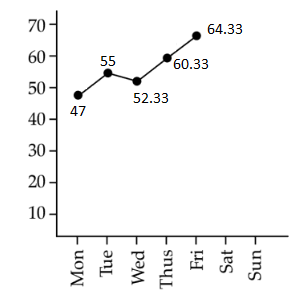# ISC Class 11 Maths Specimen Question Paper 2019 With Answers - Free Sample Paper PDF Download

ISC Class 11 Maths 2019 Specimen Question Paper with Solutions are prepared by the subject experts at BYJU’S, with detailed explanations. These ISC Class 11 Maths 2019 sample paper solutions can be accessed online as well as in a downloadable pdf format. Class 11 Students can understand all these solutions without any difficulty and they can refer to them at any time, while solving sample question papers of ISC Class 11 maths.

ISC Class 11 Students can score full marks for all the questions that appear in the exam if they practise a number of problems on each concept of ISC class 11 maths syllabus. Practising will help the students in approaching the right solutions for the questions and also quick completion of the computations.

## ISC Class 11 Maths Specimen Question Paper 2019 with Solutions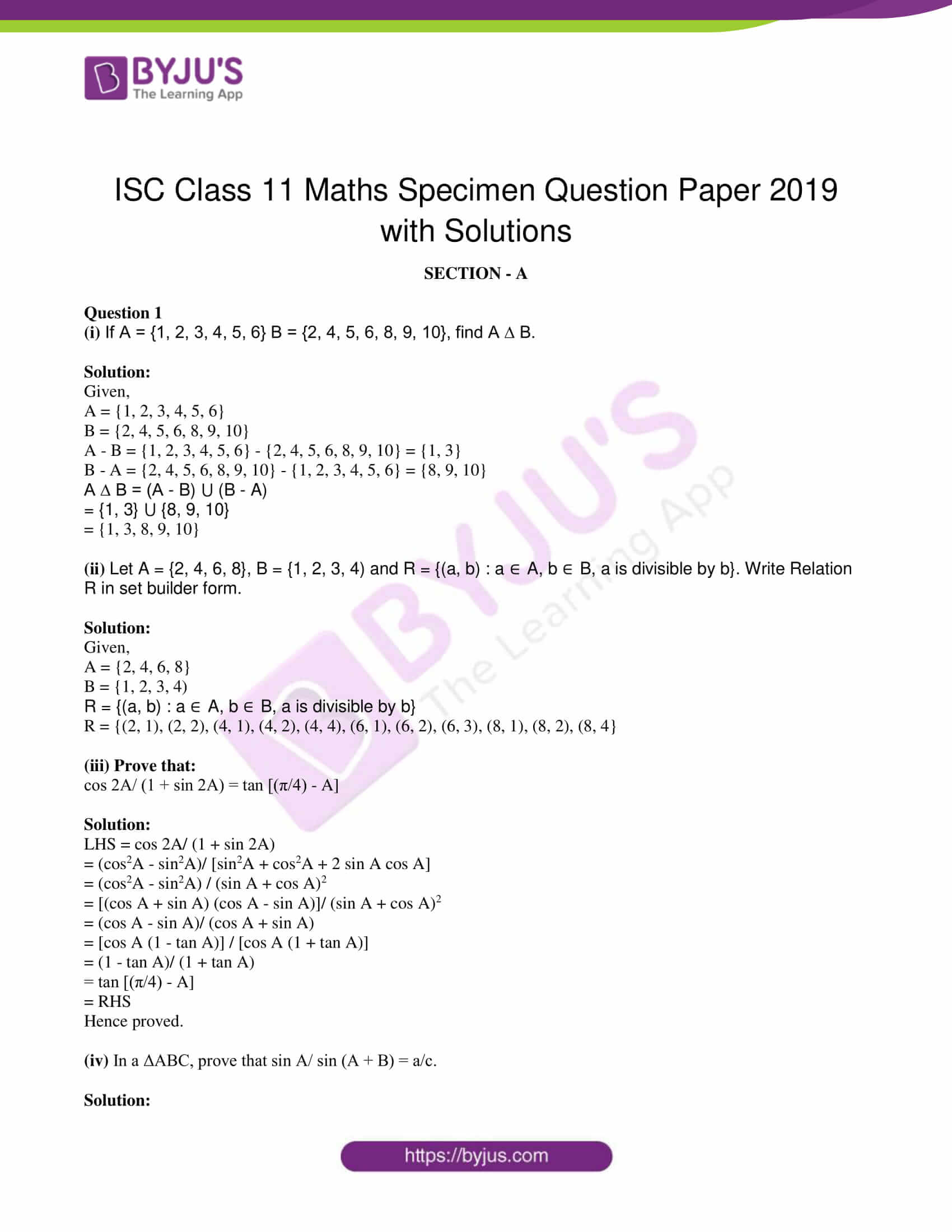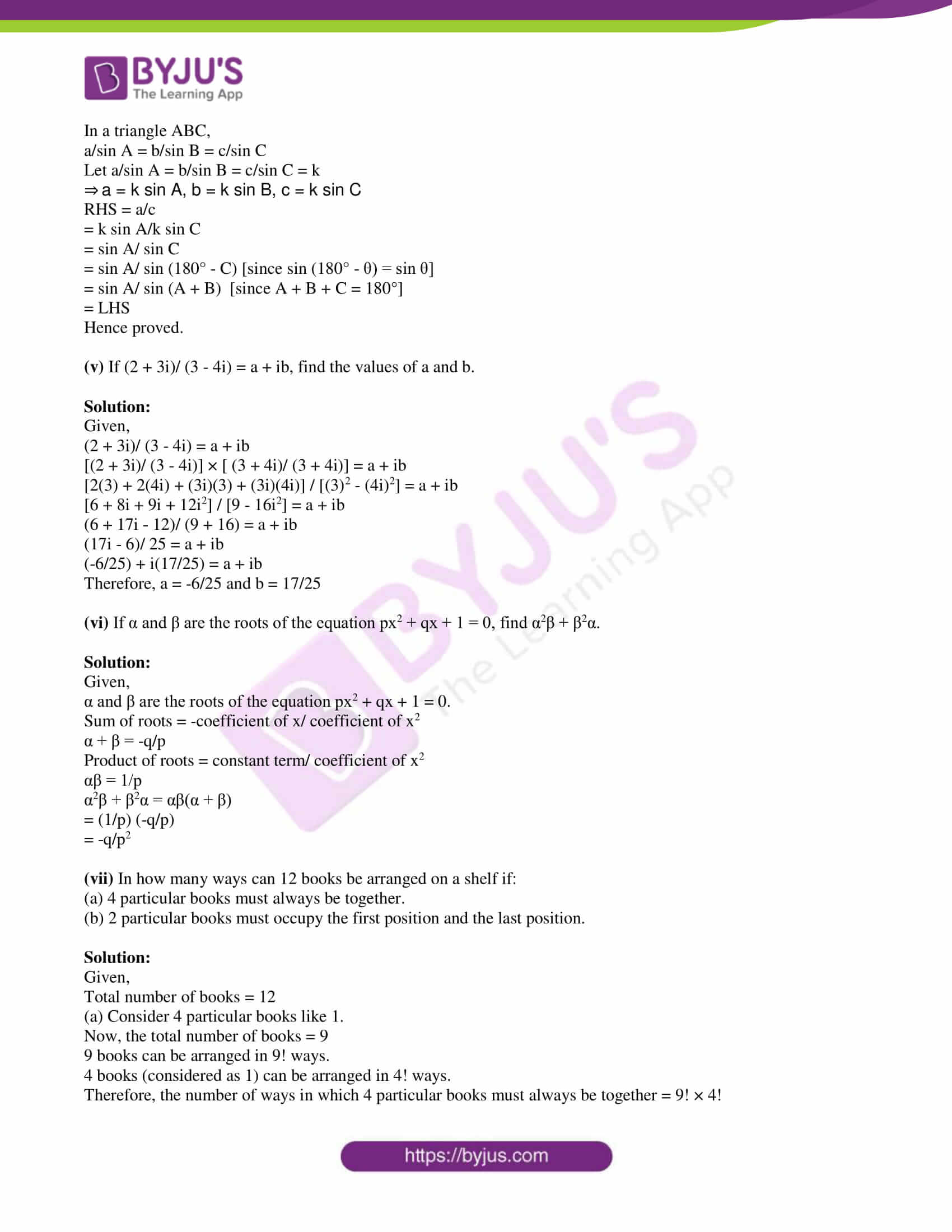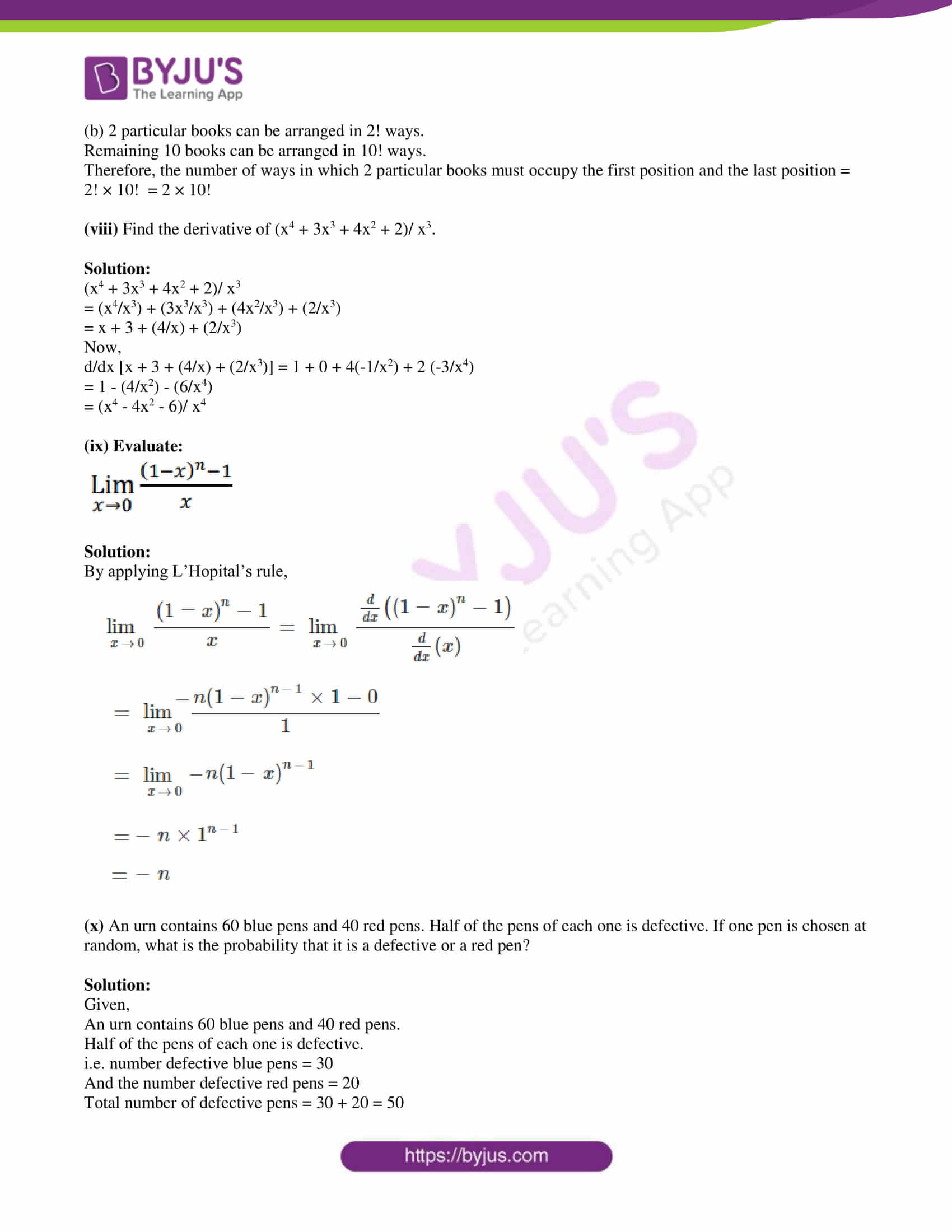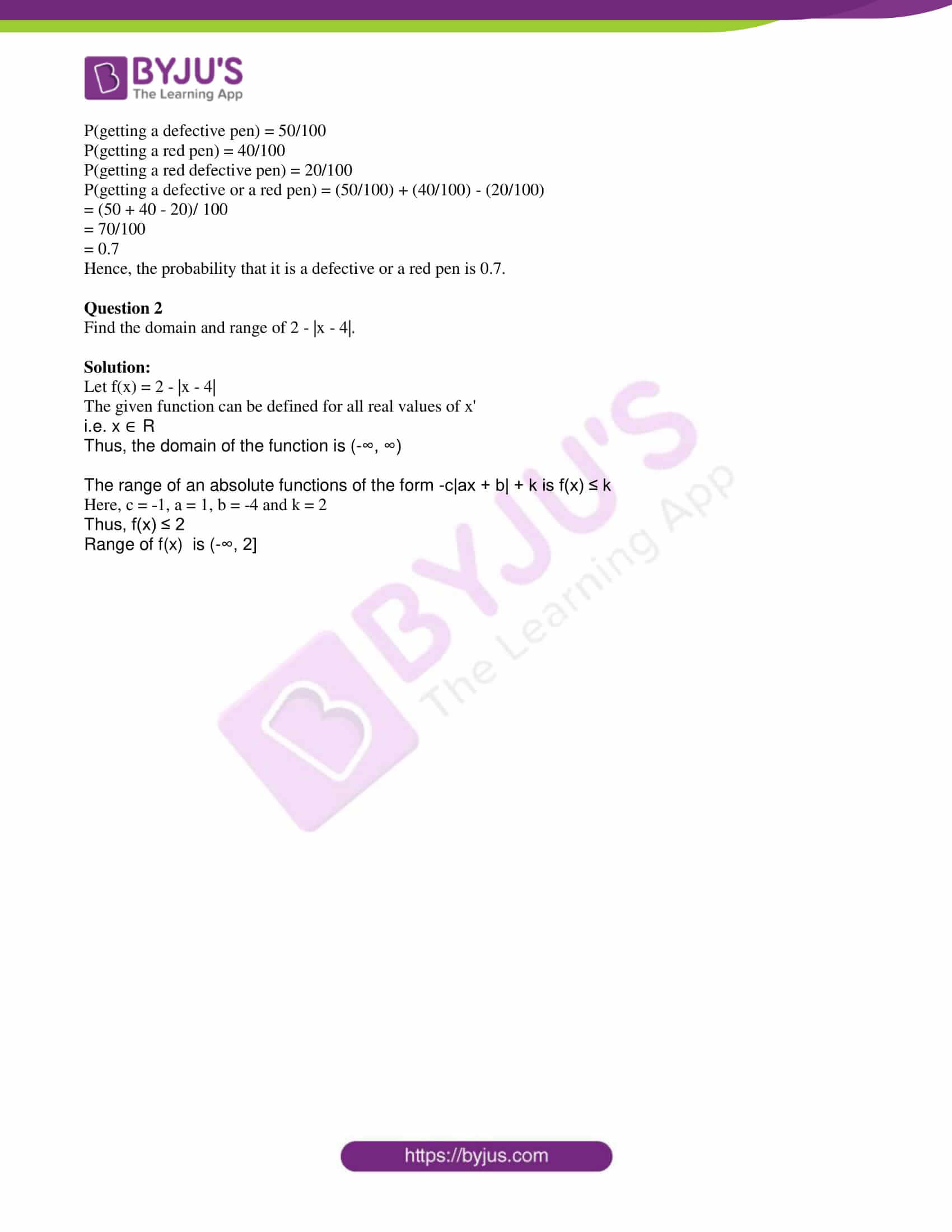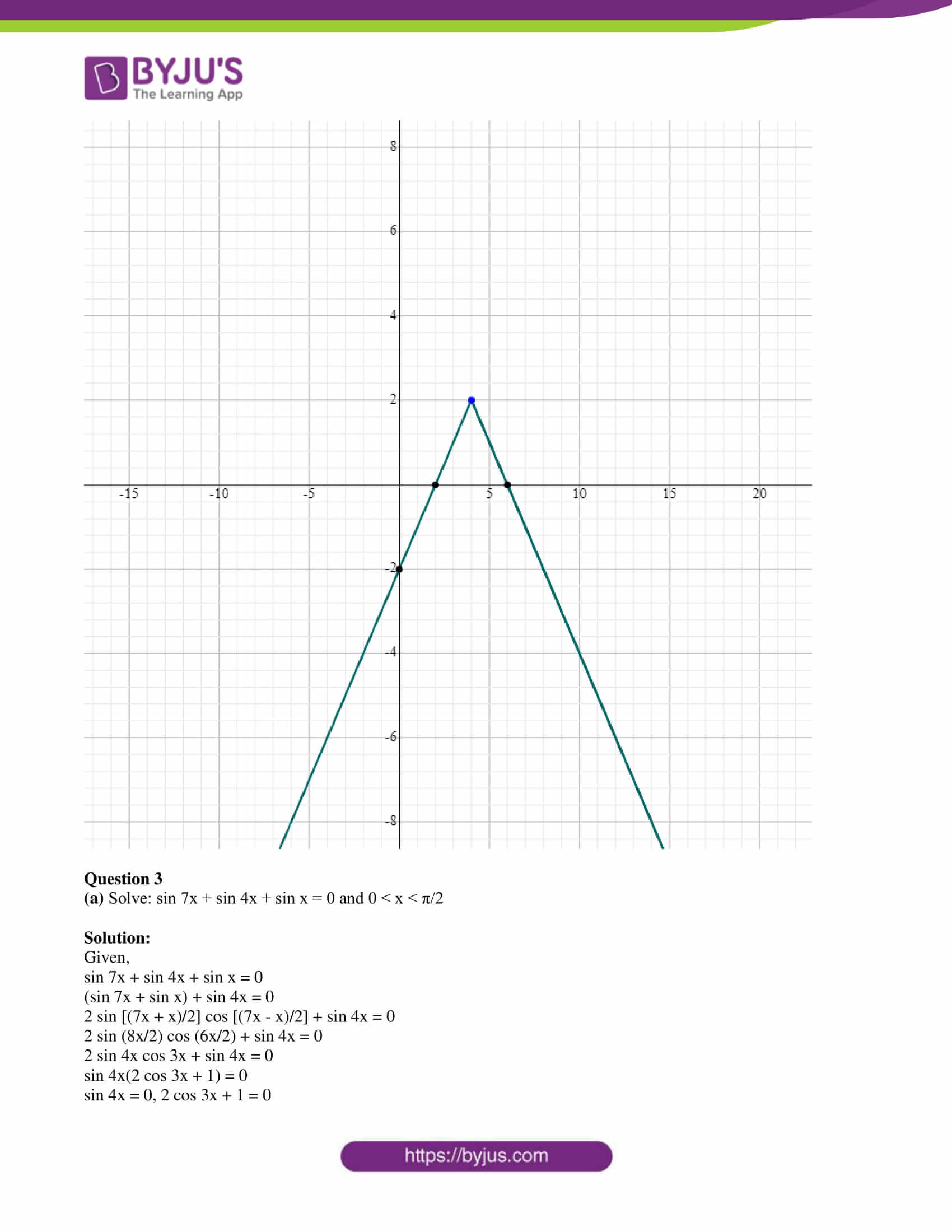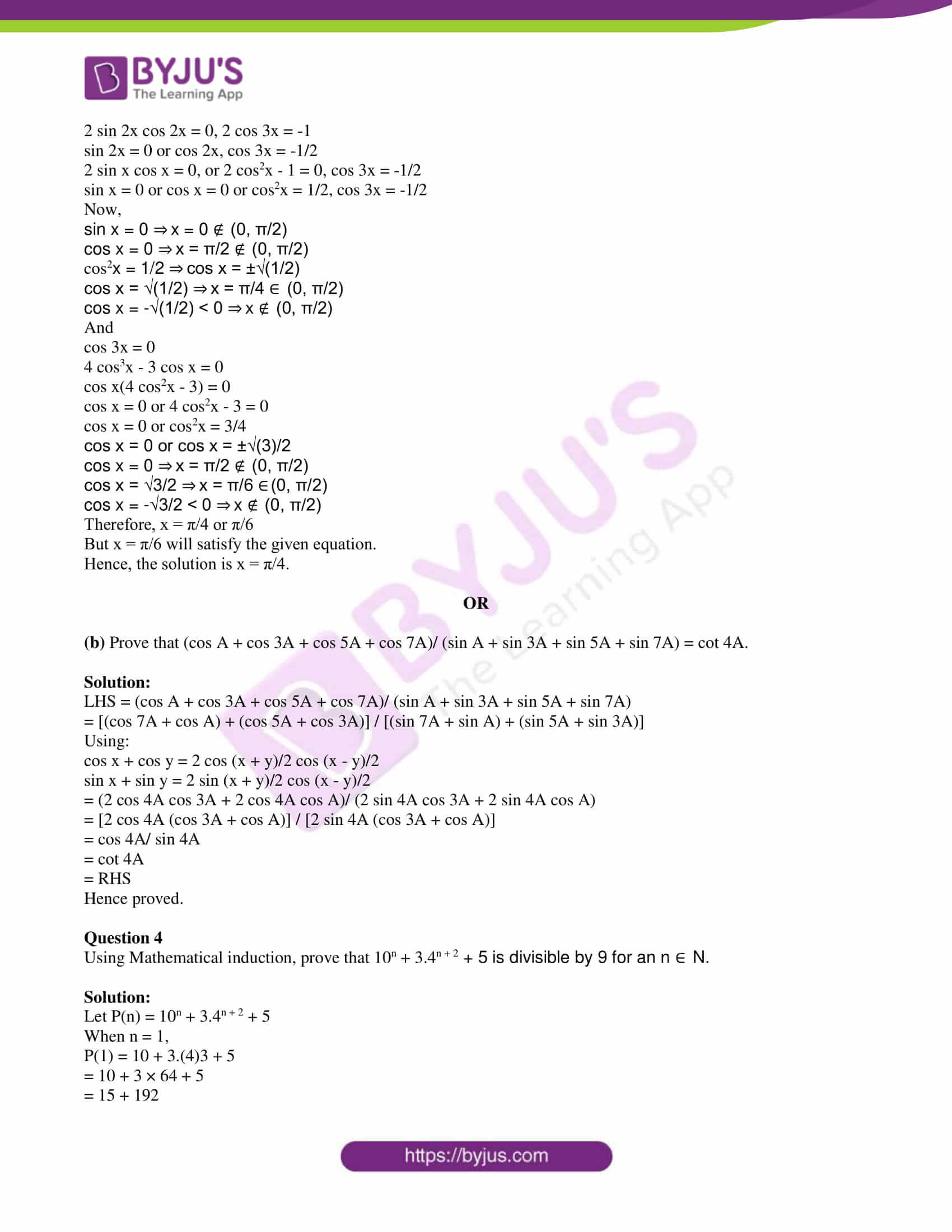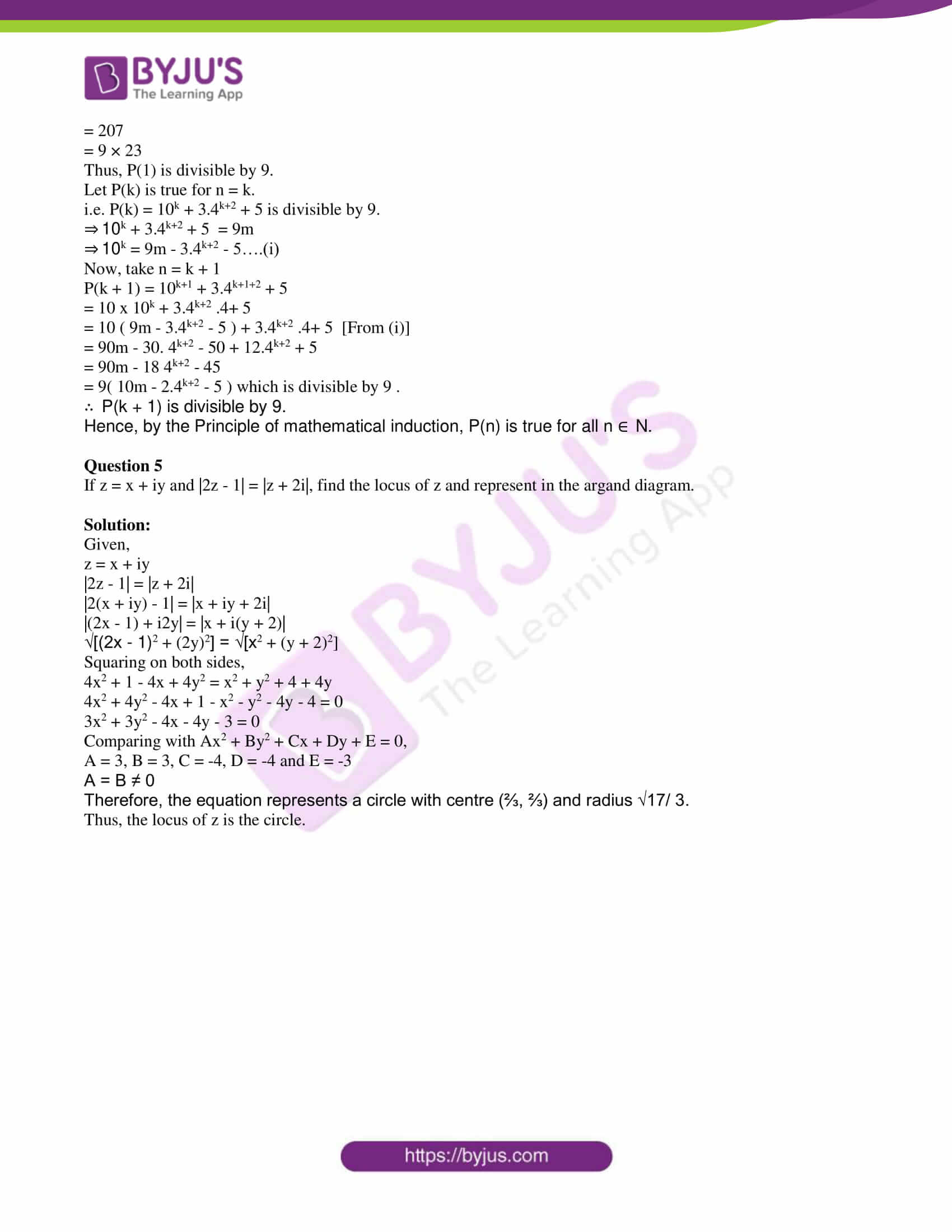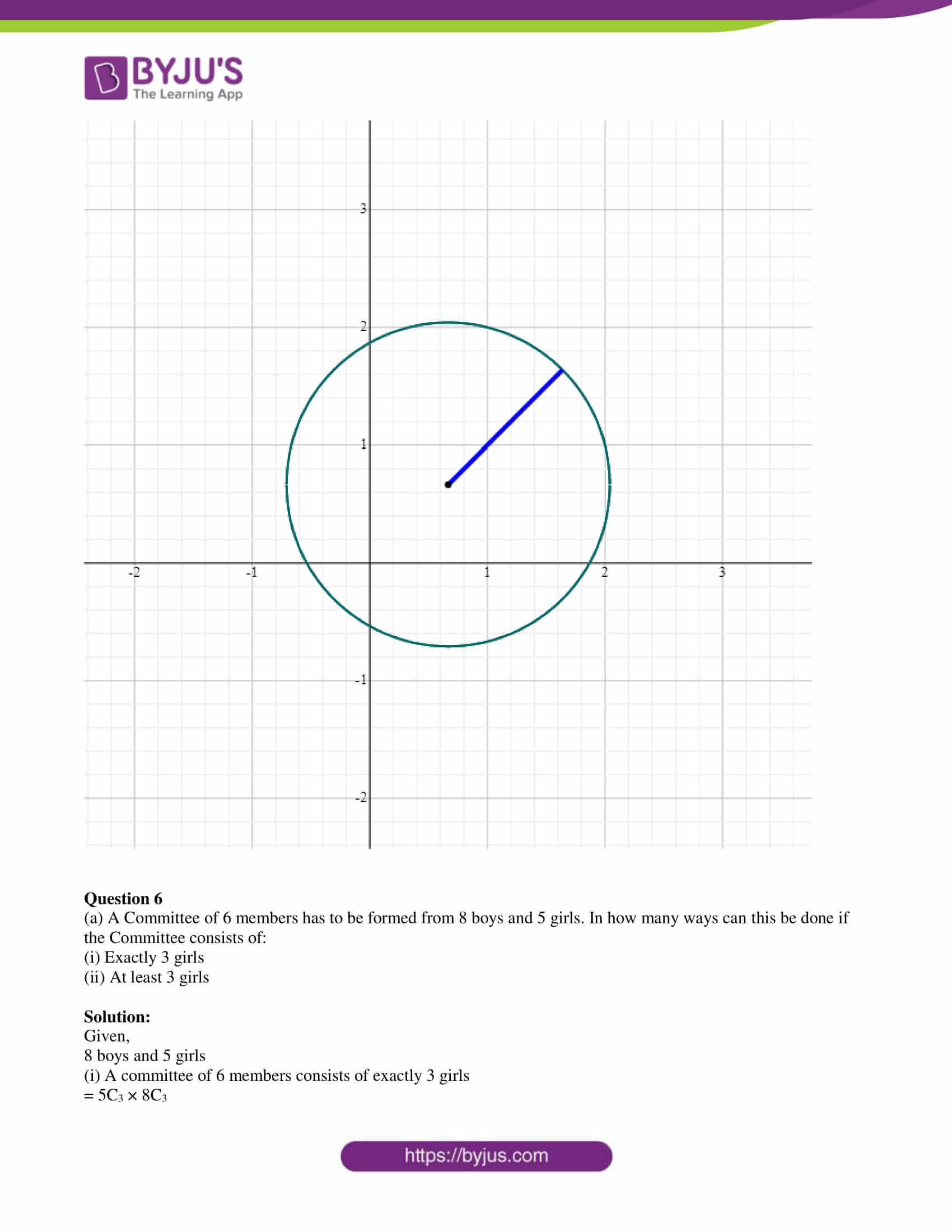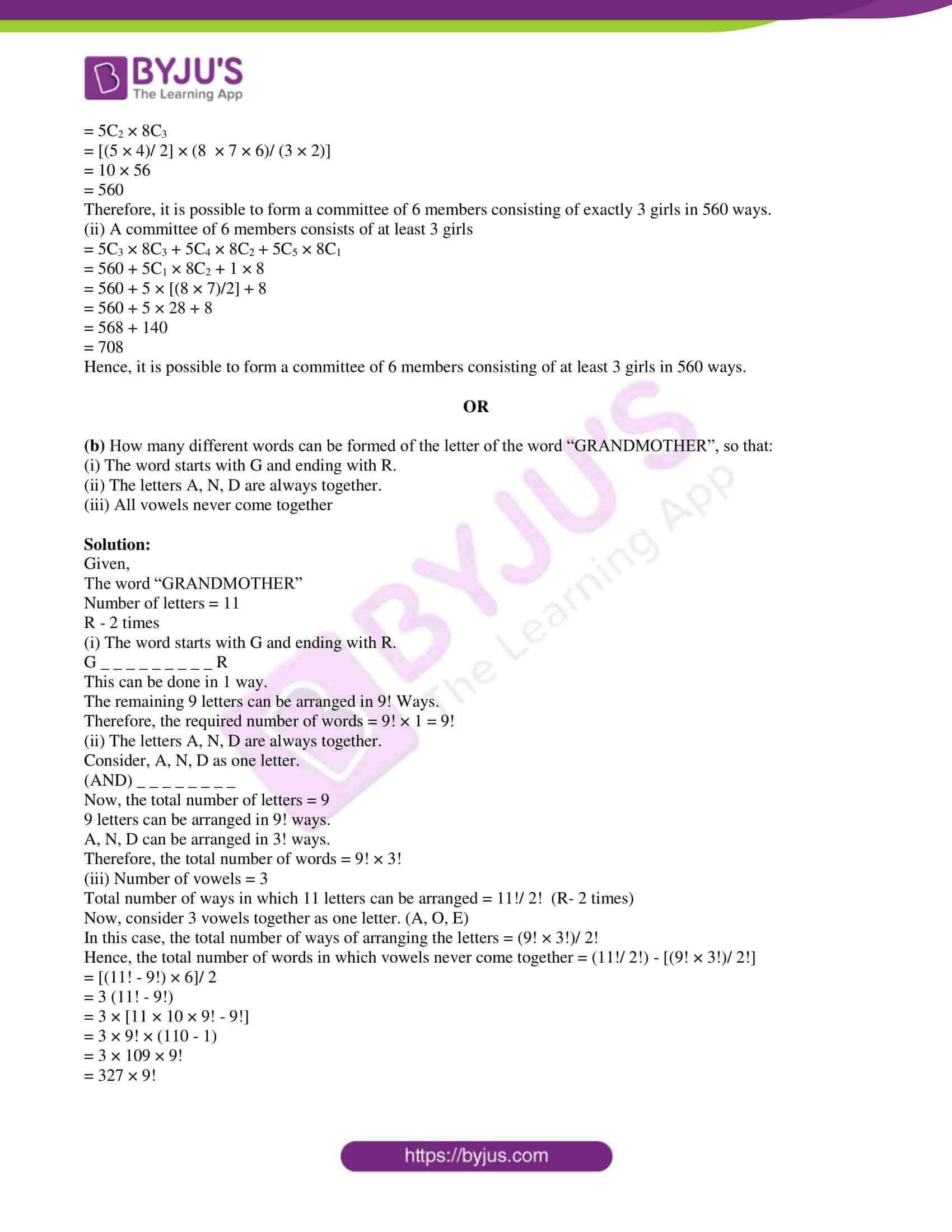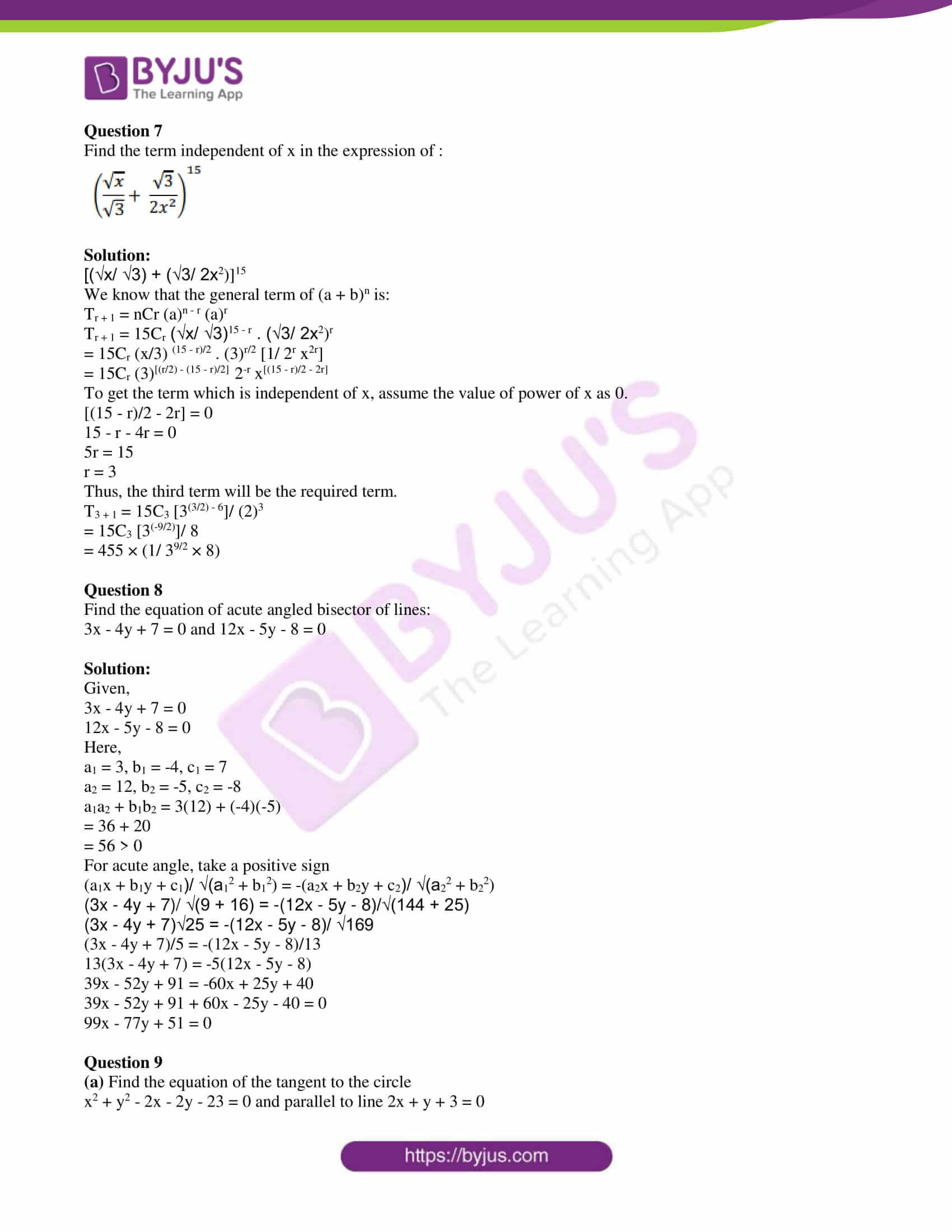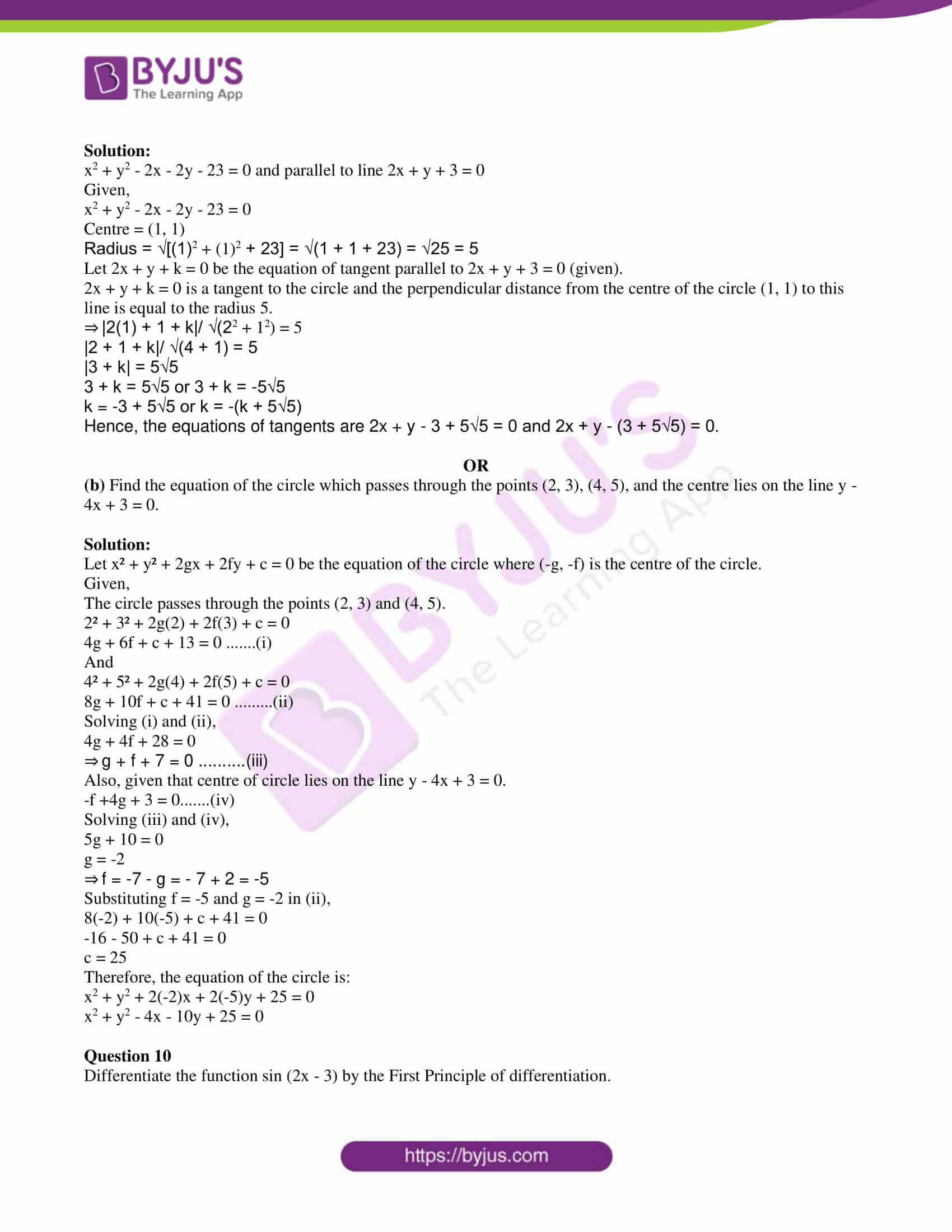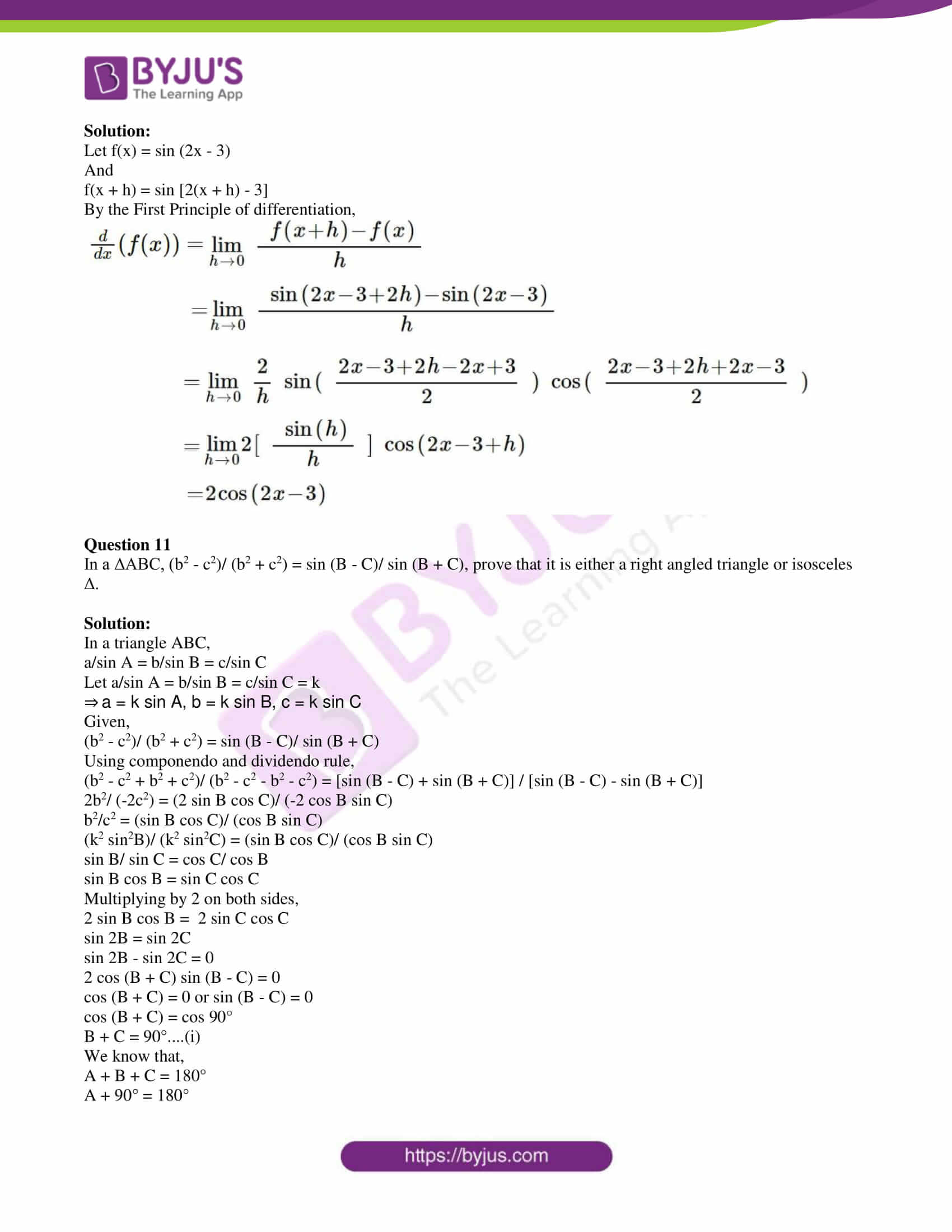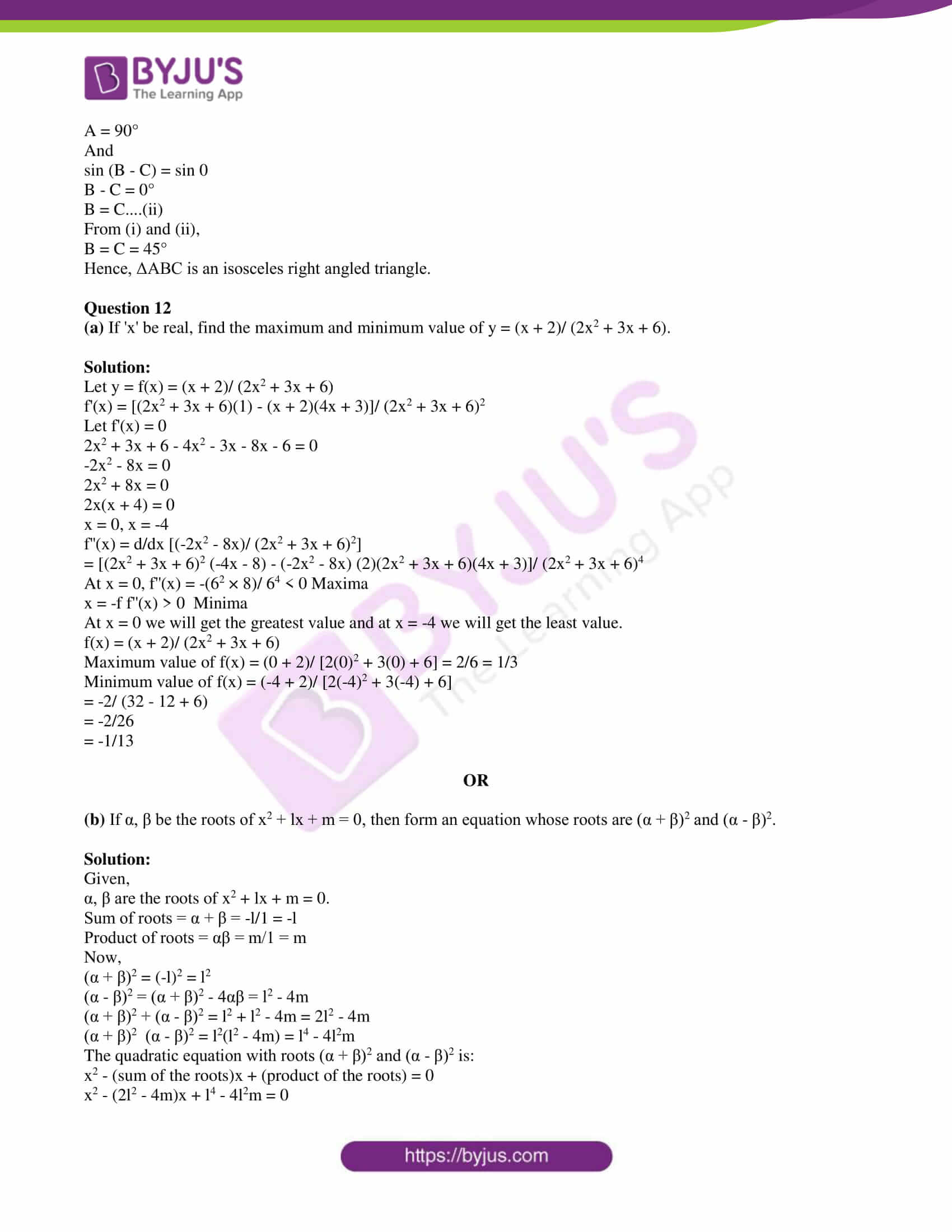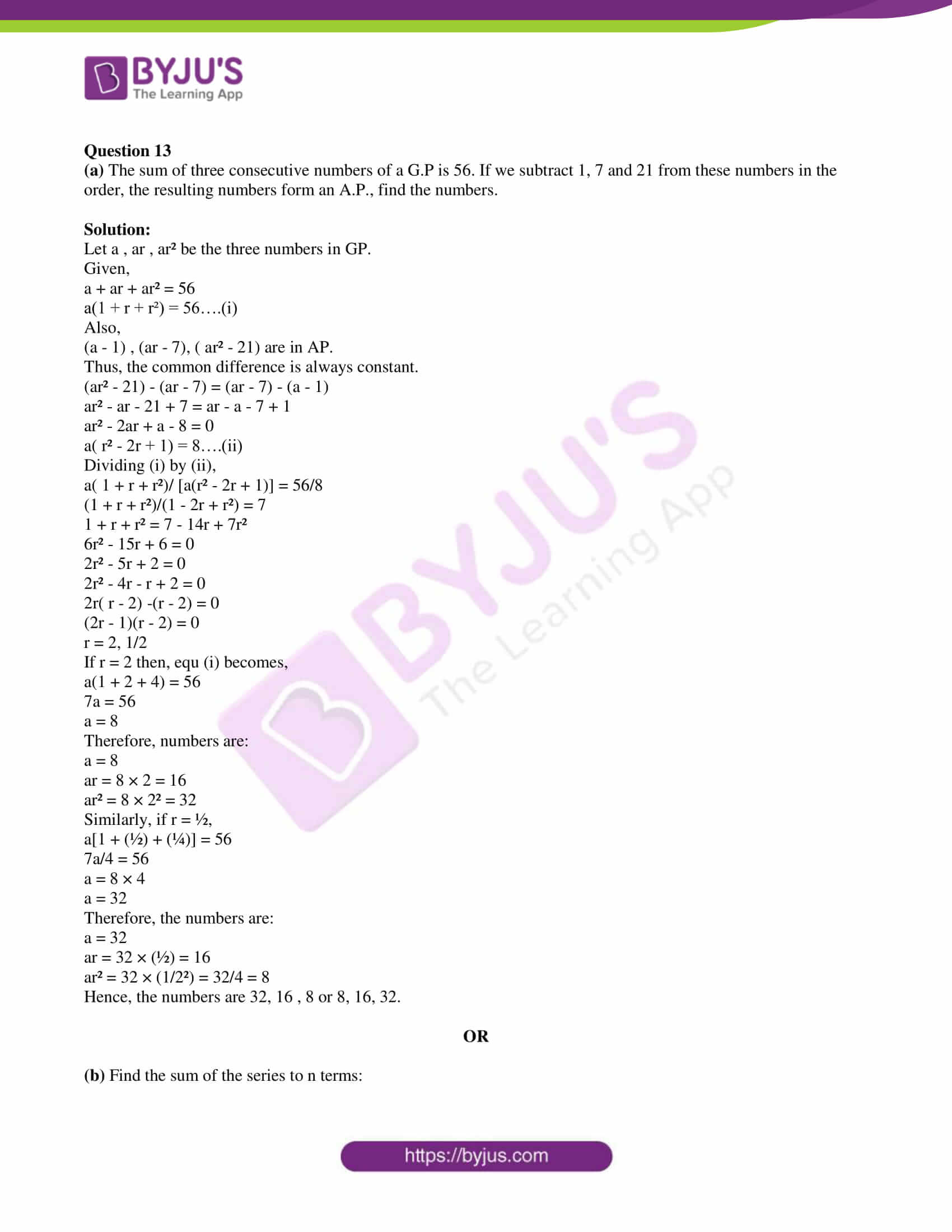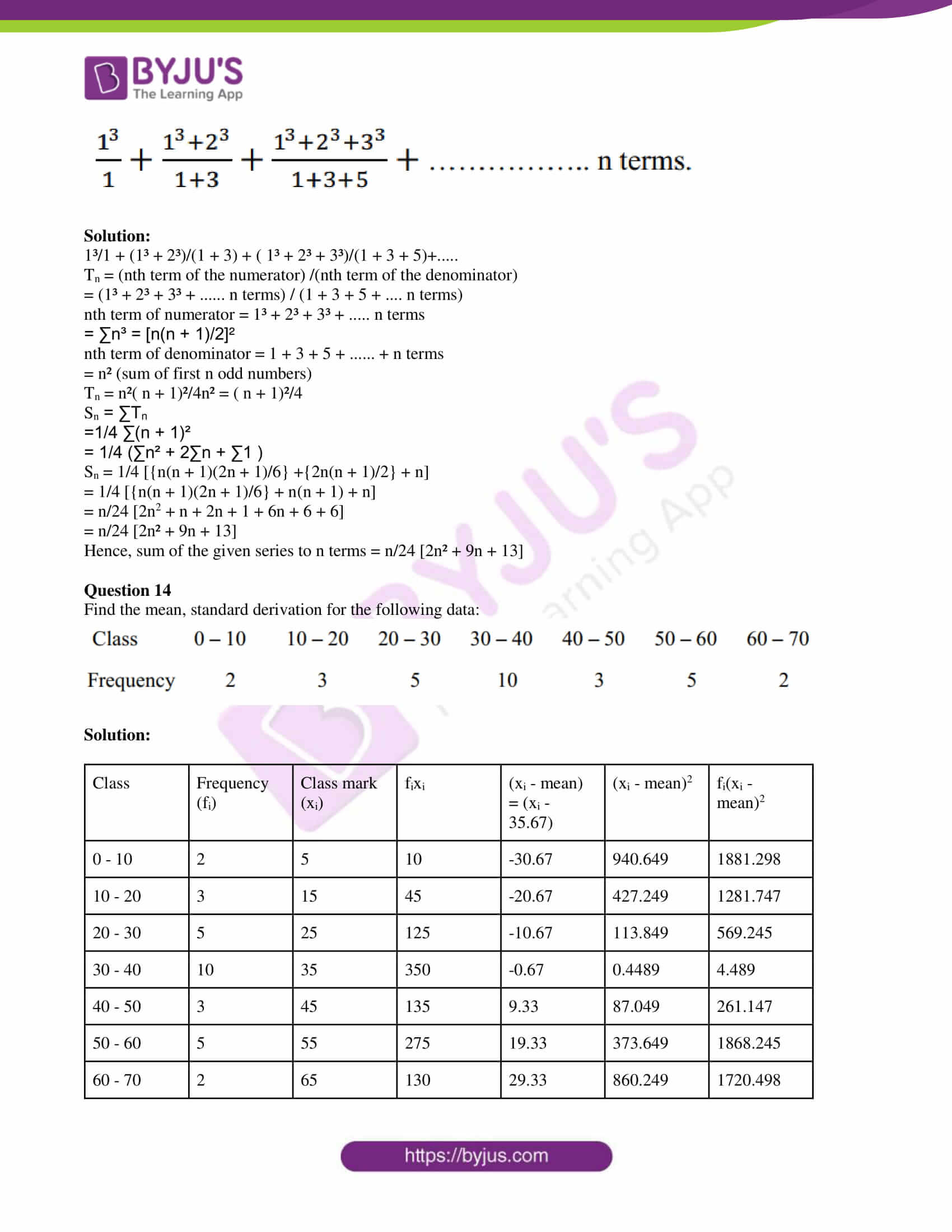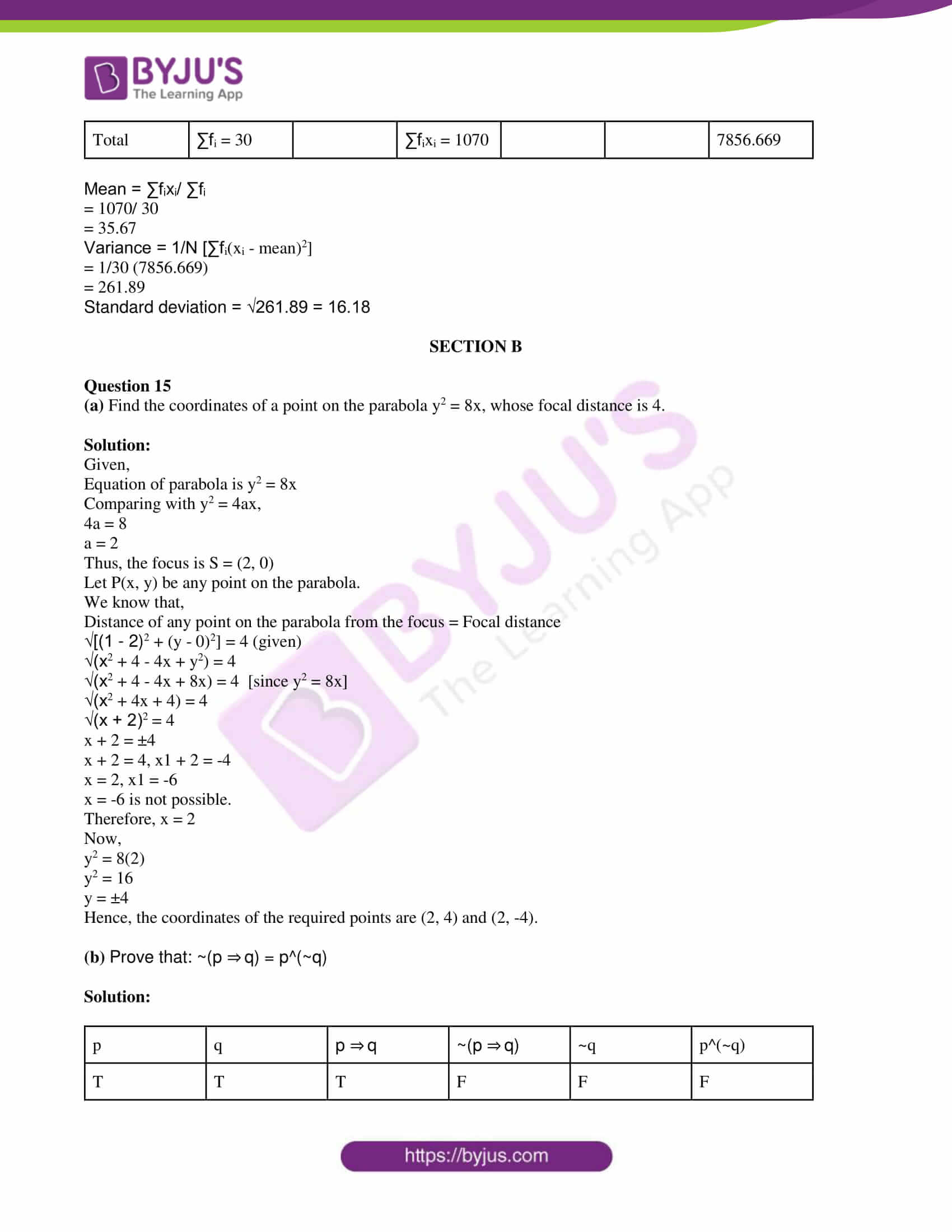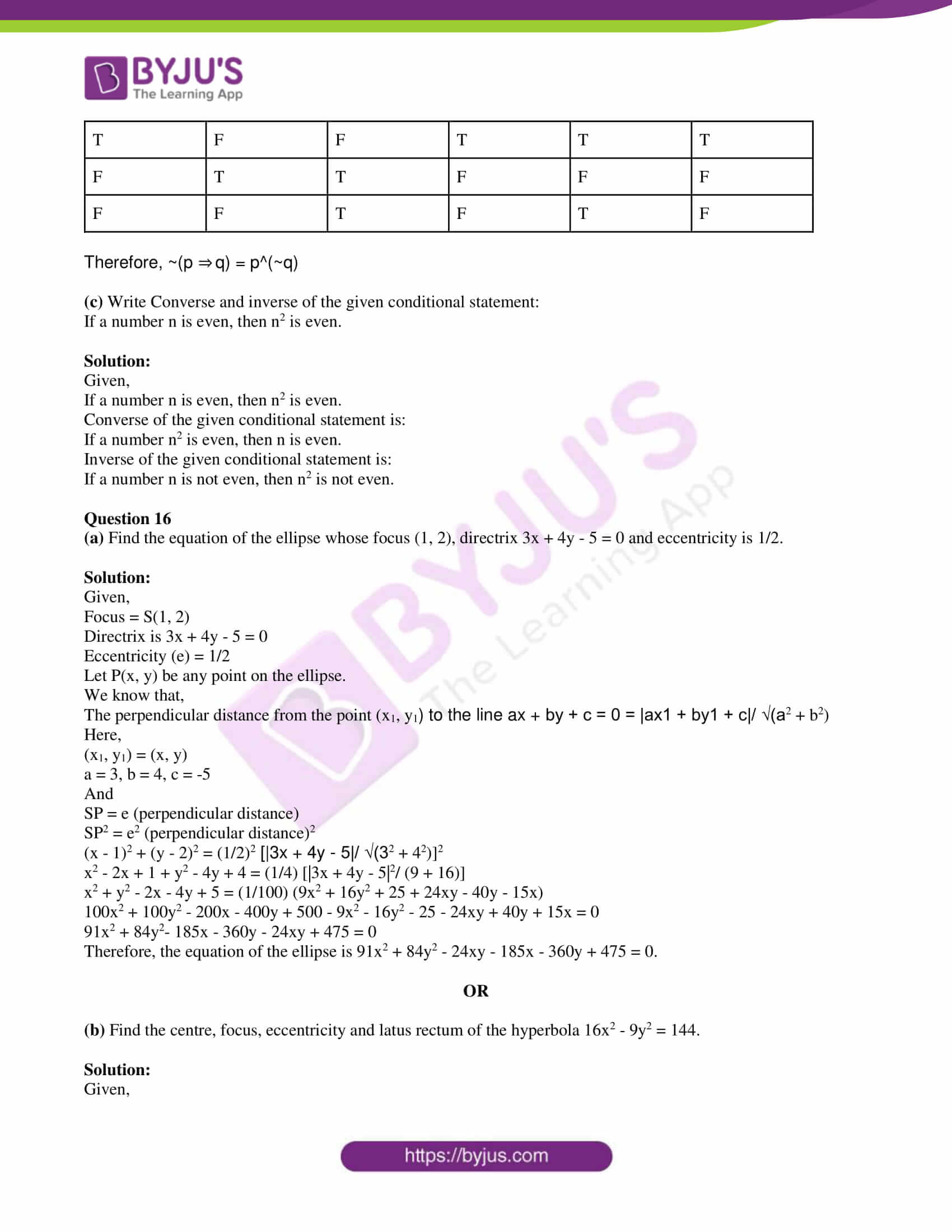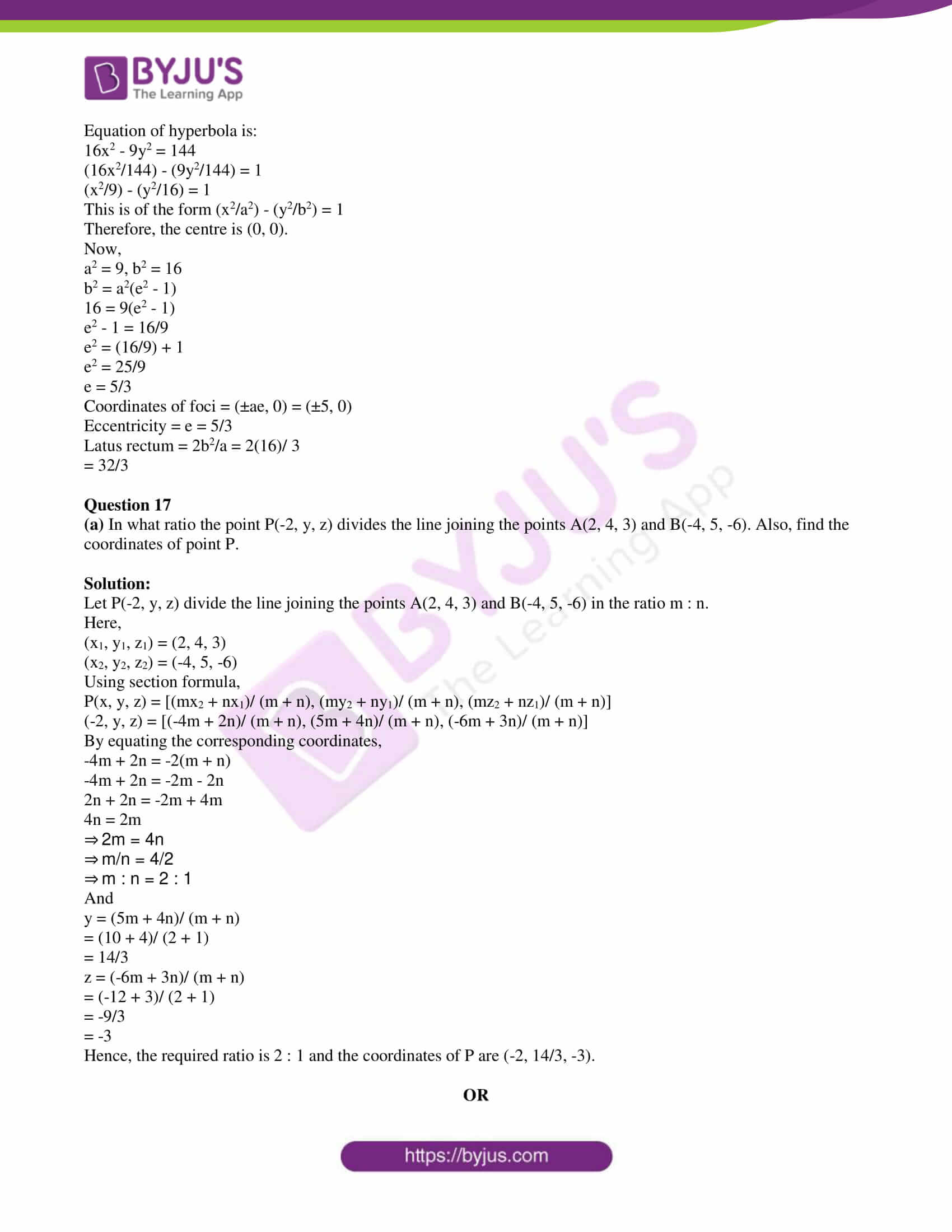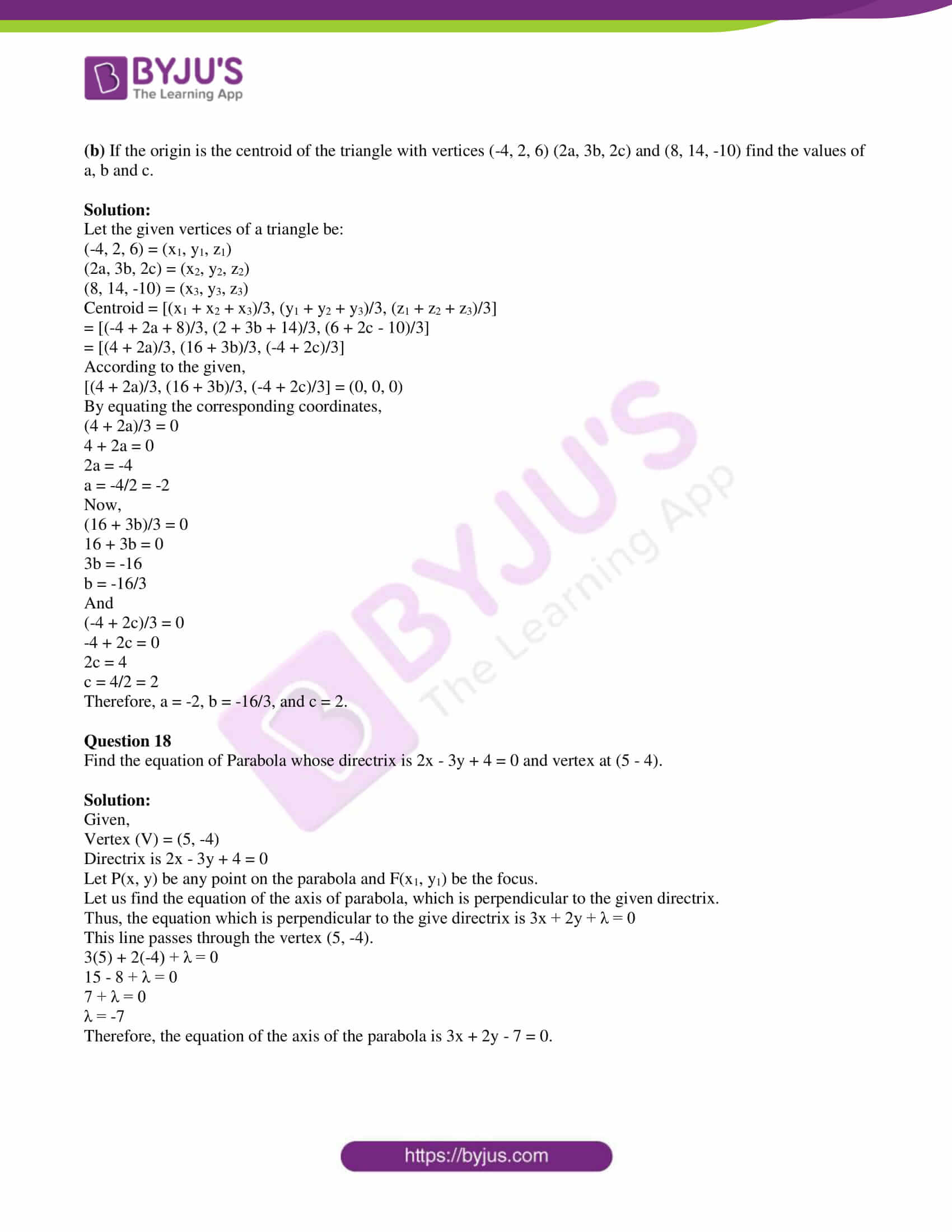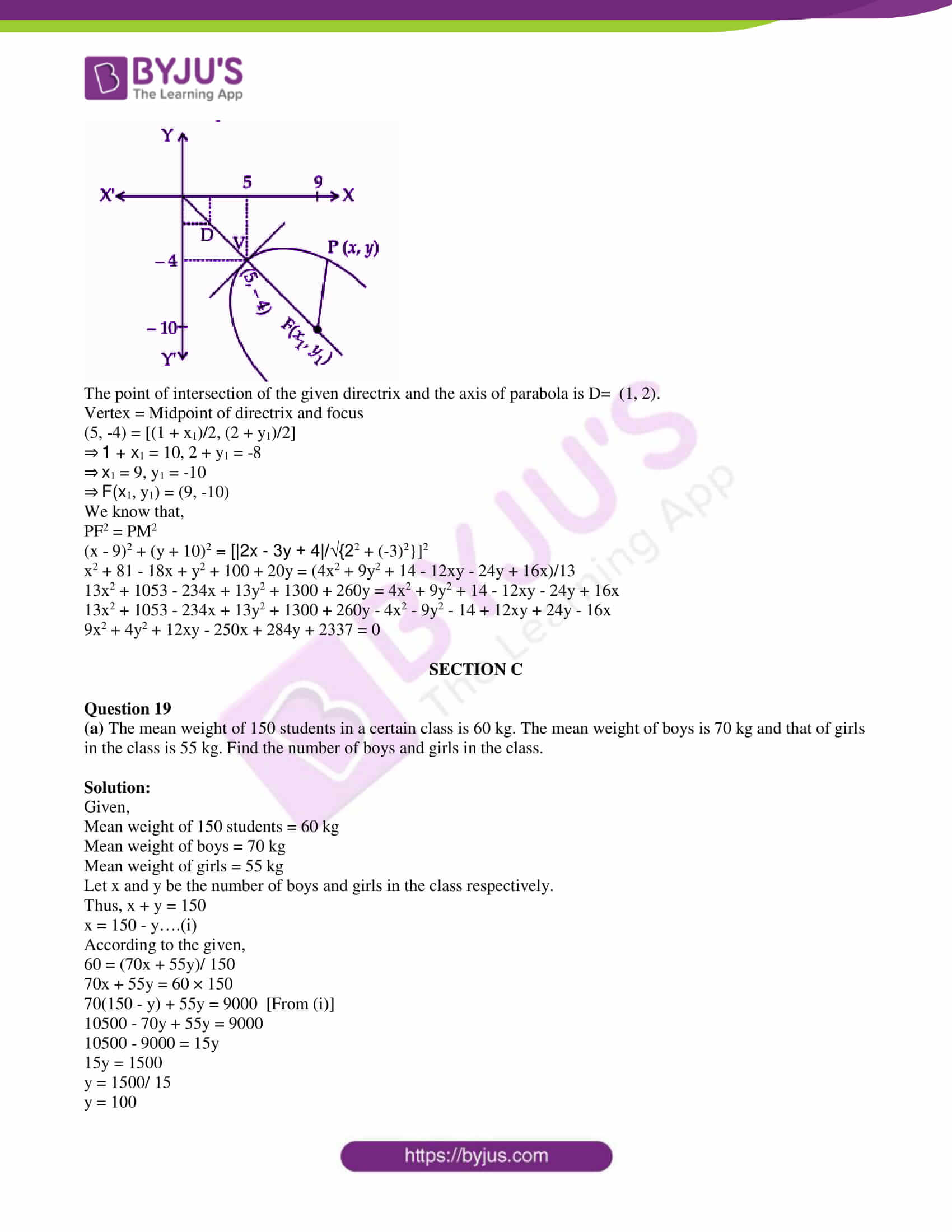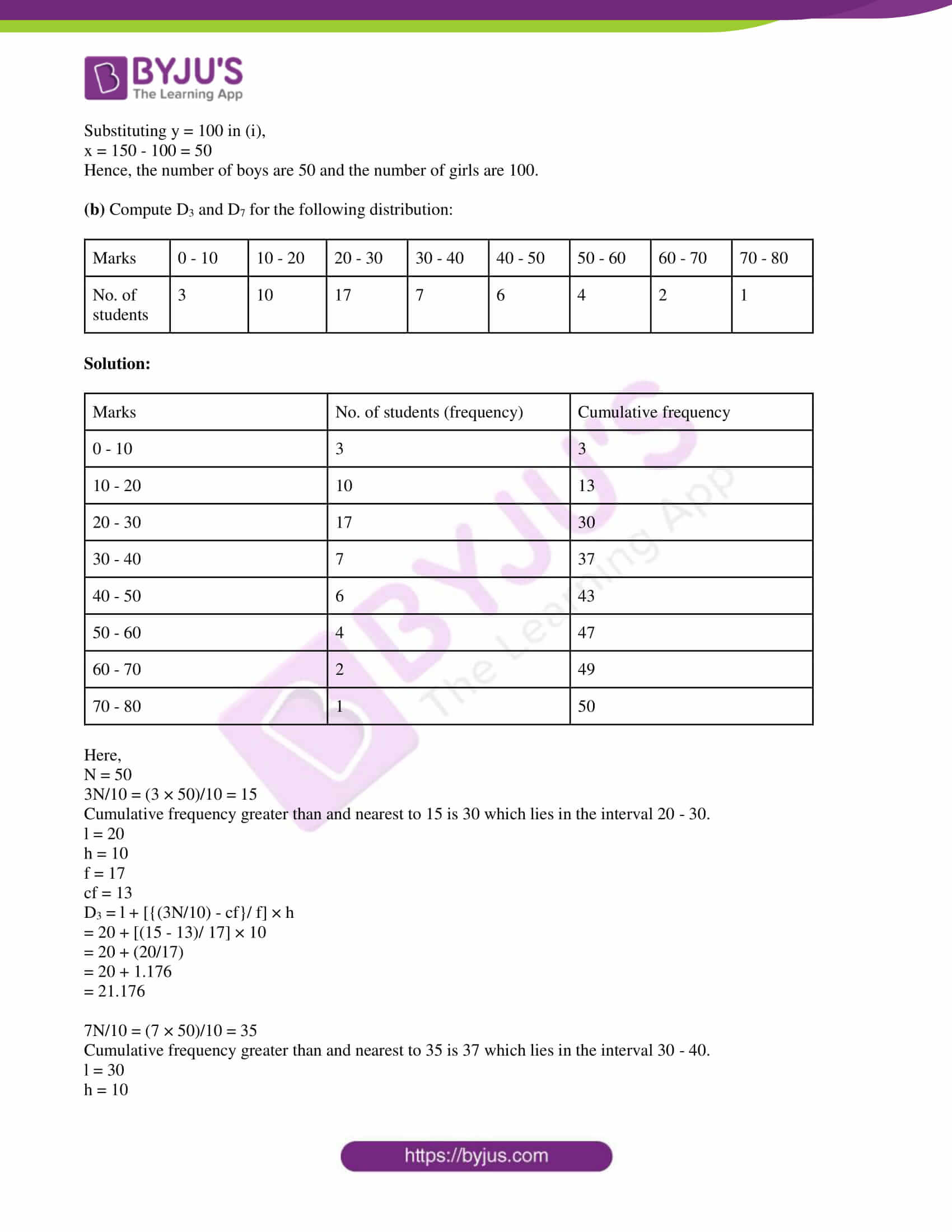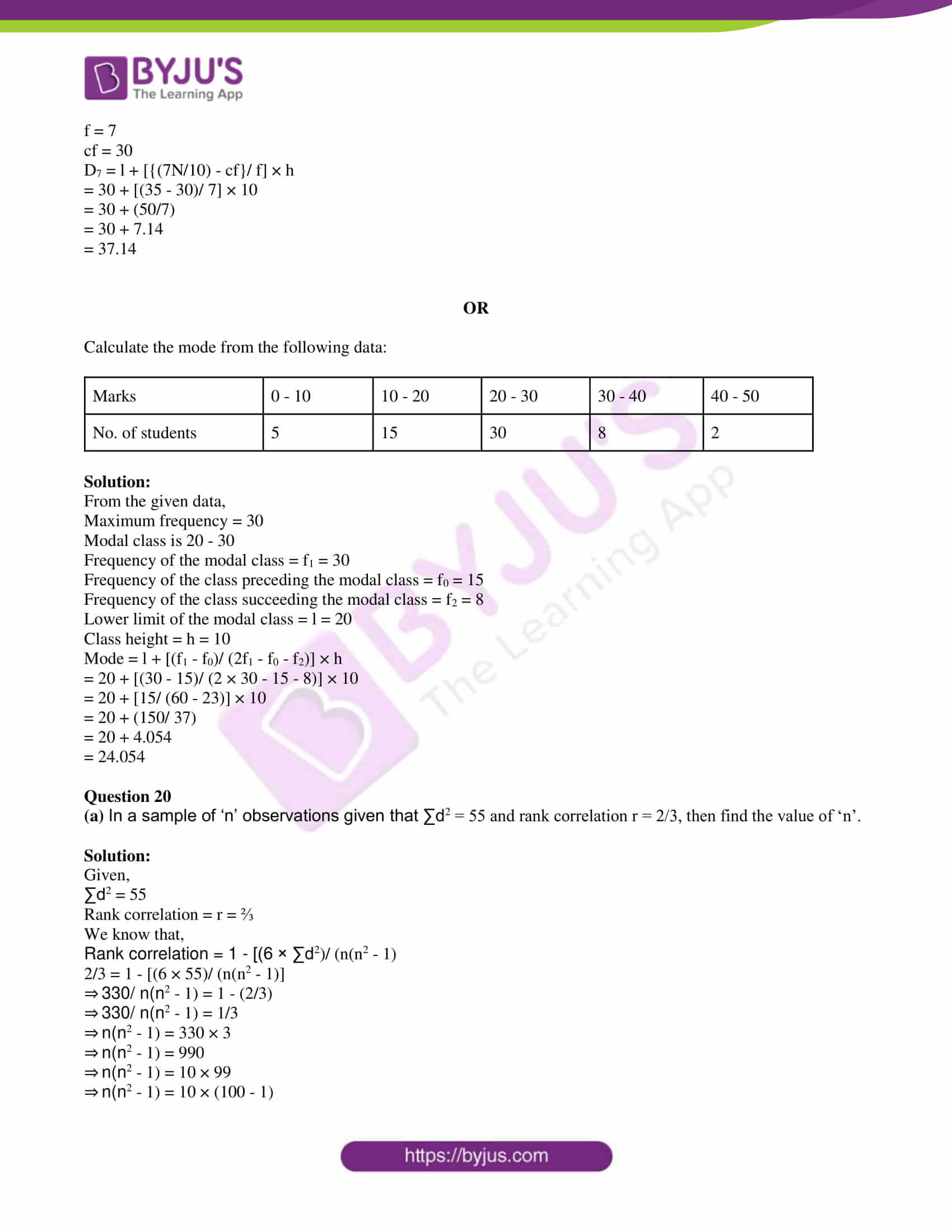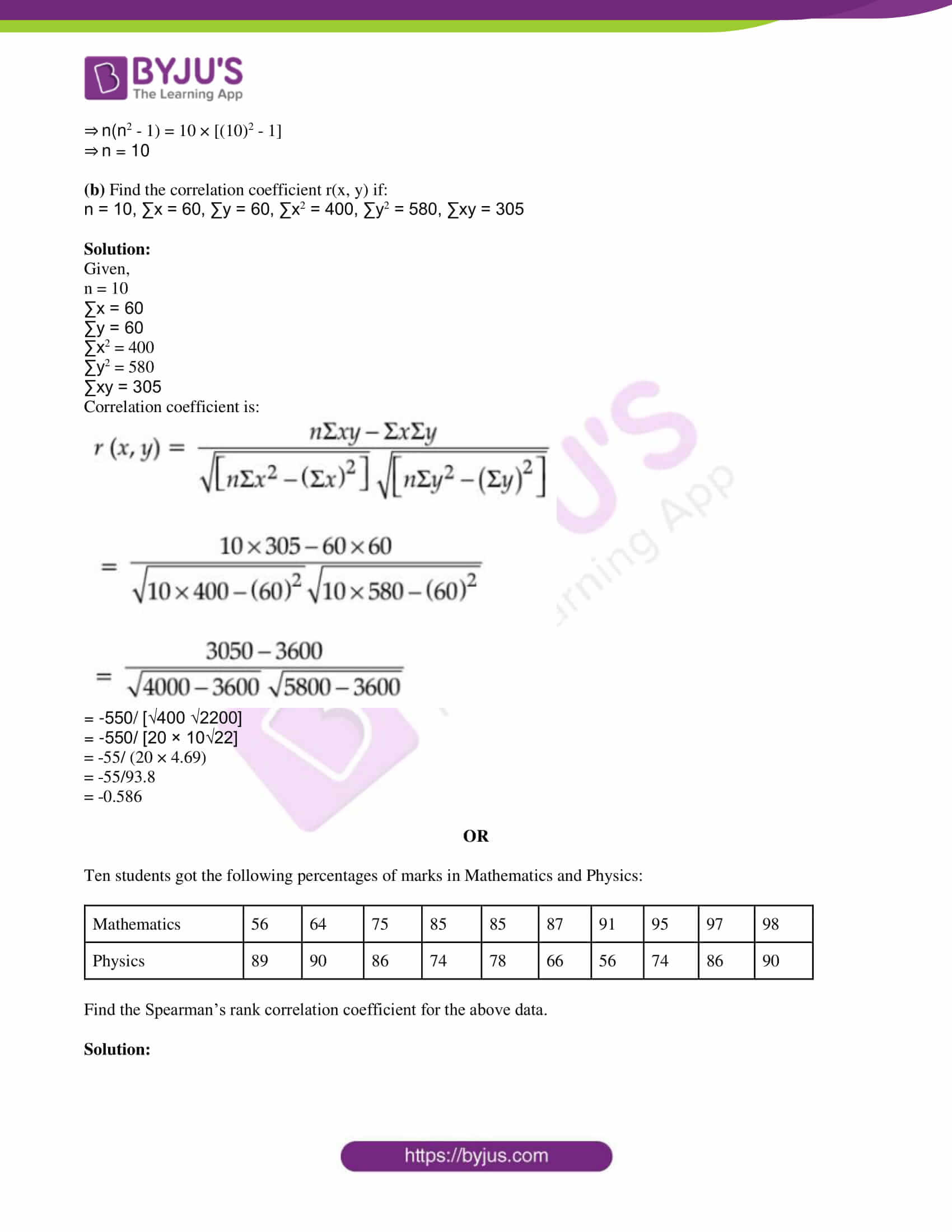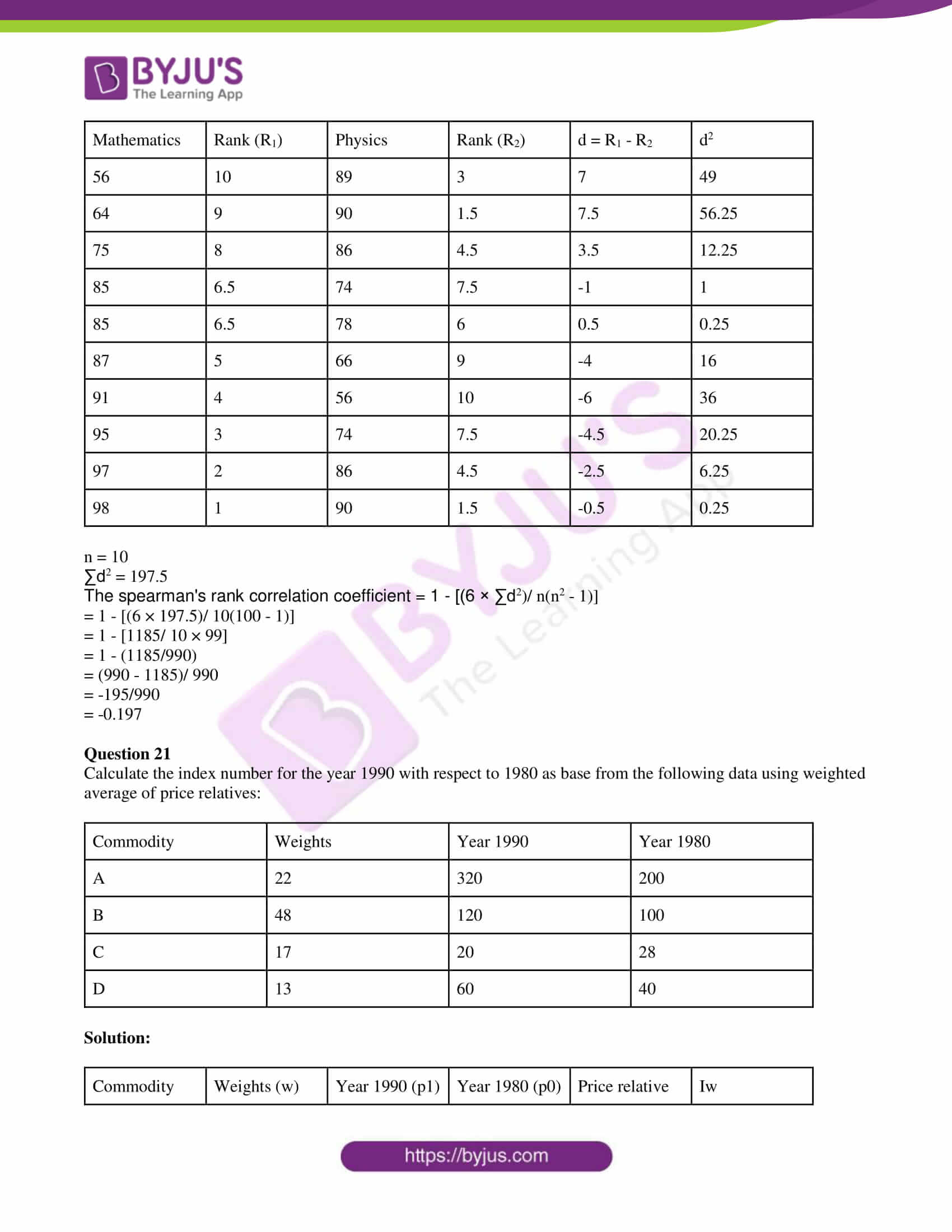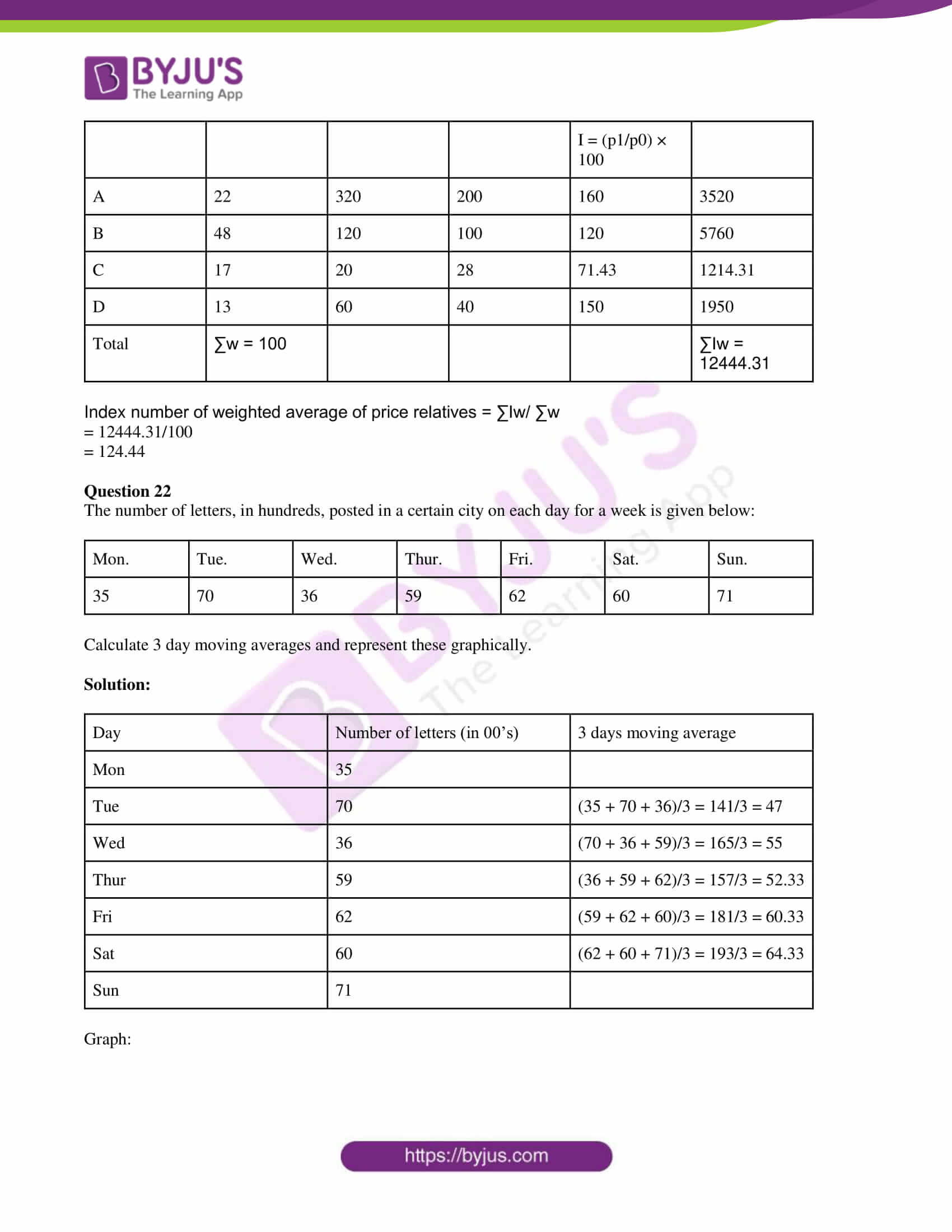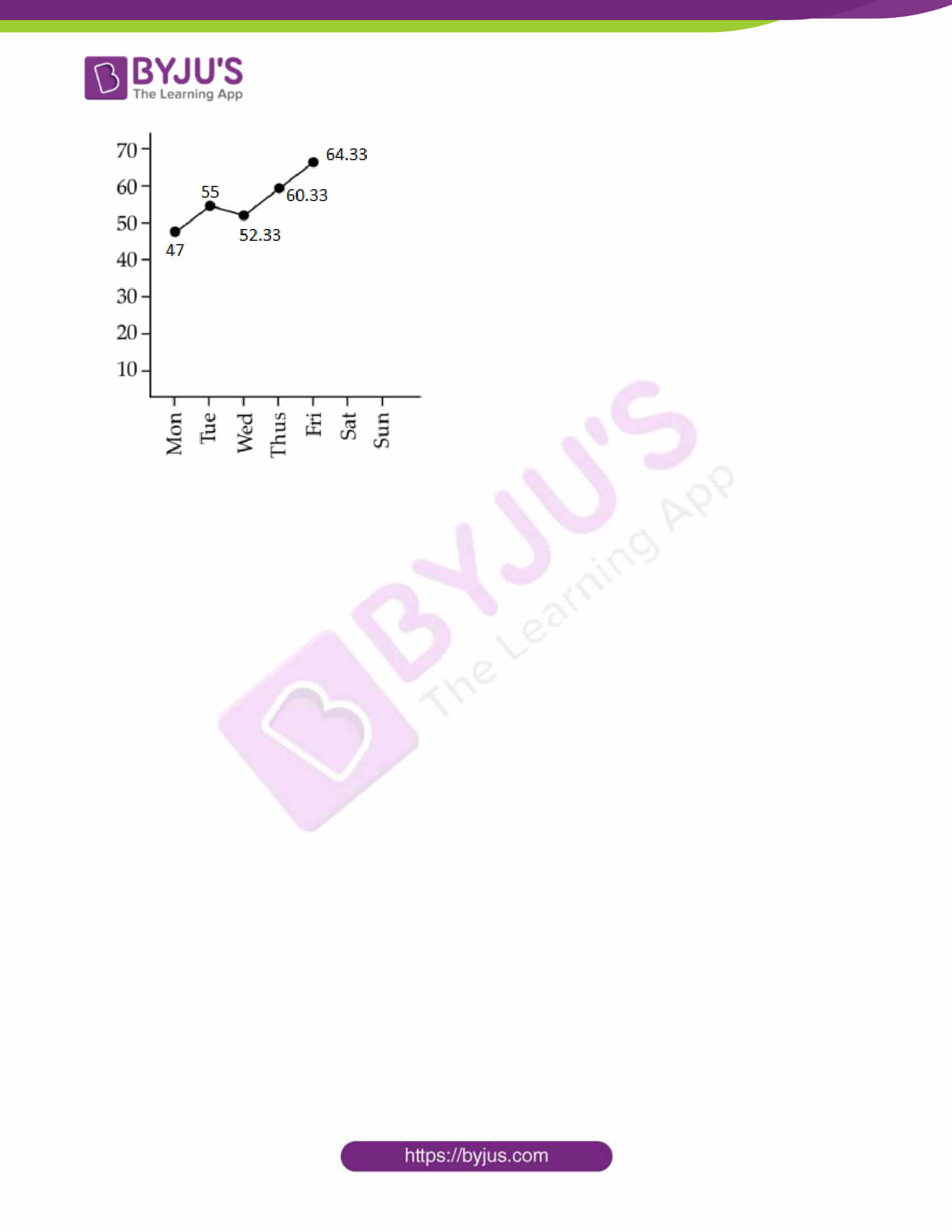SECTION – A

Question 1

(i) If A = {1, 2, 3, 4, 5, 6} B = {2, 4, 5, 6, 8, 9, 10}, find A ∆ B.

Solution:

Given,

A = {1, 2, 3, 4, 5, 6}

B = {2, 4, 5, 6, 8, 9, 10}

A – B = {1, 2, 3, 4, 5, 6} – {2, 4, 5, 6, 8, 9, 10} = {1, 3}

B – A = {2, 4, 5, 6, 8, 9, 10} – {1, 2, 3, 4, 5, 6} = {8, 9, 10}

A ∆ B = (A – B) ⋃ (B – A)

= {1, 3} ⋃ {8, 9, 10}

= {1, 3, 8, 9, 10}

(ii) Let A = {2, 4, 6, 8}, B = {1, 2, 3, 4) and R = {(a, b) : a ∈ A, b ∈ B, a is divisible by b}. Write Relation R in set builder form.

Solution:

Given,

A = {2, 4, 6, 8}

B = {1, 2, 3, 4)

R = {(a, b) : a ∈ A, b ∈ B, a is divisible by b}

R = {(2, 1), (2, 2), (4, 1), (4, 2), (4, 4), (6, 1), (6, 2), (6, 3), (8, 1), (8, 2), (8, 4}

(iii) Prove that:

cos 2A/ (1 + sin 2A) = tan [(π/4) – A]

Solution:

LHS = cos 2A/ (1 + sin 2A)

= (cos2A – sin2A)/ [sin2A + cos2A + 2 sin A cos A]

= (cos2A – sin2A) / (sin A + cos A)2

= [(cos A + sin A) (cos A – sin A)]/ (sin A + cos A)2

= (cos A – sin A)/ (cos A + sin A)

= [cos A (1 – tan A)] / [cos A (1 + tan A)]

= (1 – tan A)/ (1 + tan A)

= tan [(π/4) – A]

= RHS

Hence proved.

(iv) In a ΔABC, prove that sin A/ sin (A + B) = a/c.

Solution:

In a triangle ABC,

a/sin A = b/sin B = c/sin C

Let a/sin A = b/sin B = c/sin C = k

⇒ a = k sin A, b = k sin B, c = k sin C

RHS = a/c

= k sin A/k sin C

= sin A/ sin C

= sin A/ sin (180° – C) [since sin (180° – θ) = sin θ]

= sin A/ sin (A + B) [since A + B + C = 180°]

= LHS

Hence proved.

(v) If (2 + 3i)/ (3 – 4i) = a + ib, find the values of a and b.

Solution:

Given,

(2 + 3i)/ (3 – 4i) = a + ib

[(2 + 3i)/ (3 – 4i)] × [ (3 + 4i)/ (3 + 4i)] = a + ib

[2(3) + 2(4i) + (3i)(3) + (3i)(4i)] / [(3)2 – (4i)2] = a + ib

[6 + 8i + 9i + 12i2] / [9 – 16i2] = a + ib

(6 + 17i – 12)/ (9 + 16) = a + ib

(17i – 6)/ 25 = a + ib

(-6/25) + i(17/25) = a + ib

Therefore, a = -6/25 and b = 17/25

(vi) If α and β are the roots of the equation px2 + qx + 1 = 0, find α2β + β2α.

Solution:

Given,

α and β are the roots of the equation px2 + qx + 1 = 0.

Sum of roots = -coefficient of x/ coefficient of x2

α + β = -q/p

Product of roots = constant term/ coefficient of x2

αβ = 1/p

α2β + β2α = αβ(α + β)

= (1/p) (-q/p)

= -q/p2

(vii) In how many ways can 12 books be arranged on a shelf if:

(a) 4 particular books must always be together.

(b) 2 particular books must occupy the first position and the last position.

Solution:

Given,

Total number of books = 12

(a) Consider 4 particular books like 1.

Now, the total number of books = 9

9 books can be arranged in 9! ways.

4 books (considered as 1) can be arranged in 4! ways.

Therefore, the number of ways in which 4 particular books must always be together = 9! × 4!

(b) 2 particular books can be arranged in 2! ways.

Remaining 10 books can be arranged in 10! ways.

Therefore, the number of ways in which 2 particular books must occupy the first position and the last position = 2! × 10! = 2 × 10!

(viii) Find the derivative of (x4 + 3x3 + 4x2 + 2)/ x3.

Solution:

(x4 + 3x3 + 4x2 + 2)/ x3

= (x4/x3) + (3x3/x3) + (4x2/x3) + (2/x3)

= x + 3 + (4/x) + (2/x3)

Now,

d/dx [x + 3 + (4/x) + (2/x3)] = 1 + 0 + 4(-1/x2) + 2 (-3/x4)

= 1 – (4/x2) – (6/x4)

= (x4 – 4x2 – 6)/ x4

(ix) Evaluate: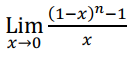Solution:

By applying L’Hopital’s rule,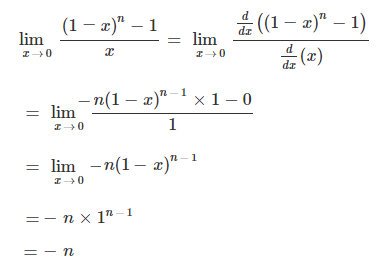(x) An urn contains 60 blue pens and 40 red pens. Half of the pens of each one is defective. If one pen is chosen at random, what is the probability that it is a defective or a red pen?

Solution:

Given,

An urn contains 60 blue pens and 40 red pens.

Half of the pens of each one is defective.

i.e. number defective blue pens = 30

And the number defective red pens = 20

Total number of defective pens = 30 + 20 = 50

P(getting a defective pen) = 50/100

P(getting a red pen) = 40/100

P(getting a red defective pen) = 20/100

P(getting a defective or a red pen) = (50/100) + (40/100) – (20/100)

= (50 + 40 – 20)/ 100

= 70/100

= 0.7

Hence, the probability that it is a defective or a red pen is 0.7.

Question 2

Find the domain and range of 2 – |x – 4|.

Solution:

Let f(x) = 2 – |x – 4|

The given function can be defined for all real values of x’

i.e. x ∈ R

Thus, the domain of the function is (-∞, ∞)

The range of an absolute functions of the form -c|ax + b| + k is f(x) ≤ k

Here, c = -1, a = 1, b = -4 and k = 2

Thus, f(x) ≤ 2

Range of f(x) is (-∞, 2]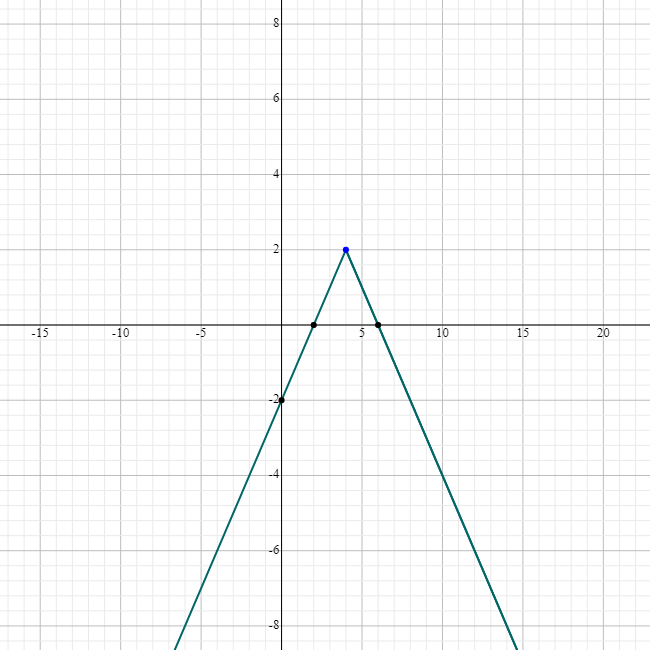Question 3

(a) Solve: sin 7x + sin 4x + sin x = 0 and 0 < x < π/2

Solution:

Given,

sin 7x + sin 4x + sin x = 0

(sin 7x + sin x) + sin 4x = 0

2 sin [(7x + x)/2] cos [(7x – x)/2] + sin 4x = 0

2 sin (8x/2) cos (6x/2) + sin 4x = 0

2 sin 4x cos 3x + sin 4x = 0

sin 4x(2 cos 3x + 1) = 0

sin 4x = 0, 2 cos 3x + 1 = 0

2 sin 2x cos 2x = 0, 2 cos 3x = -1

sin 2x = 0 or cos 2x, cos 3x = -1/2

2 sin x cos x = 0, or 2 cos2x – 1 = 0, cos 3x = -1/2

sin x = 0 or cos x = 0 or cos2x = 1/2, cos 3x = -1/2

Now,

sin x = 0 ⇒ x = 0 ∉ (0, π/2)

cos x = 0 ⇒ x = π/2 ∉ (0, π/2)

cos2x = 1/2 ⇒ cos x = ±√(1/2)

cos x = √(1/2) ⇒ x = π/4 ∈ (0, π/2)

cos x = -√(1/2) < 0 ⇒ x ∉ (0, π/2)

And

cos 3x = 0

4 cos3x – 3 cos x = 0

cos x(4 cos2x – 3) = 0

cos x = 0 or 4 cos2x – 3 = 0

cos x = 0 or cos2x = 3/4

cos x = 0 or cos x = ±√(3)/2

cos x = 0 ⇒ x = π/2 ∉ (0, π/2)

cos x = √3/2 ⇒ x = π/6 ∈(0, π/2)

cos x = -√3/2 < 0 ⇒ x ∉ (0, π/2)

Therefore, x = π/4 or π/6

But x = π/6 will satisfy the given equation.

Hence, the solution is x = π/4.

OR

(b) Prove that (cos A + cos 3A + cos 5A + cos 7A)/ (sin A + sin 3A + sin 5A + sin 7A) = cot 4A.

Solution:

LHS = (cos A + cos 3A + cos 5A + cos 7A)/ (sin A + sin 3A + sin 5A + sin 7A)

= [(cos 7A + cos A) + (cos 5A + cos 3A)] / [(sin 7A + sin A) + (sin 5A + sin 3A)]

Using:

cos x + cos y = 2 cos (x + y)/2 cos (x – y)/2

sin x + sin y = 2 sin (x + y)/2 cos (x – y)/2

= (2 cos 4A cos 3A + 2 cos 4A cos A)/ (2 sin 4A cos 3A + 2 sin 4A cos A)

= [2 cos 4A (cos 3A + cos A)] / [2 sin 4A (cos 3A + cos A)]

= cos 4A/ sin 4A

= cot 4A

= RHS

Hence proved.

Question 4

Using Mathematical induction, prove that 10n + 3.4n + 2 + 5 is divisible by 9 for an n ∈ N.

Solution:

Let P(n) = 10n + 3.4n + 2 + 5

When n = 1,

P(1) = 10 + 3.(4)3 + 5

= 10 + 3 × 64 + 5

= 15 + 192

= 207

= 9 × 23

Thus, P(1) is divisible by 9.

Let P(k) is true for n = k.

i.e. P(k) = 10k + 3.4k+2 + 5 is divisible by 9.

⇒ 10k + 3.4k+2 + 5 = 9m

⇒ 10k = 9m – 3.4k+2 – 5….(i)

Now, take n = k + 1

P(k + 1) = 10k+1 + 3.4k+1+2 + 5

= 10 x 10k + 3.4k+2 .4+ 5

= 10 ( 9m – 3.4k+2 – 5 ) + 3.4k+2 .4+ 5 [From (i)]

= 90m – 30. 4k+2 – 50 + 12.4k+2 + 5

= 90m – 18 4k+2 – 45

= 9( 10m – 2.4k+2 – 5 ) which is divisible by 9 .

∴ P(k + 1) is divisible by 9.

Hence, by the Principle of mathematical induction, P(n) is true for all n ∈ N.

Question 5

If z = x + iy and |2z – 1| = |z + 2i|, find the locus of z and represent in the argand diagram.

Solution:

Given,

z = x + iy

|2z – 1| = |z + 2i|

|2(x + iy) – 1| = |x + iy + 2i|

|(2x – 1) + i2y| = |x + i(y + 2)|

√[(2x – 1)2 + (2y)2] = √[x2 + (y + 2)2]

Squaring on both sides,

4x2 + 1 – 4x + 4y2 = x2 + y2 + 4 + 4y

4x2 + 4y2 – 4x + 1 – x2 – y2 – 4y – 4 = 0

3x2 + 3y2 – 4x – 4y – 3 = 0

Comparing with Ax2 + By2 + Cx + Dy + E = 0,

A = 3, B = 3, C = -4, D = -4 and E = -3

A = B ≠ 0

Therefore, the equation represents a circle with centre (⅔, ⅔) and radius √17/ 3.

Thus, the locus of z is the circle.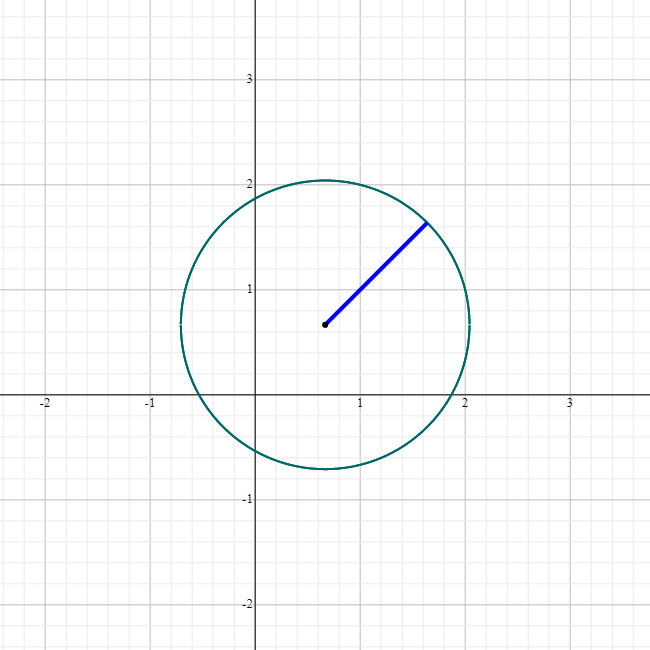Question 6

(a) A Committee of 6 members has to be formed from 8 boys and 5 girls. In how many ways can this be done if the Committee consists of:

(i) Exactly 3 girls

(ii) At least 3 girls

Solution:

Given,

8 boys and 5 girls

(i) A committee of 6 members consists of exactly 3 girls

= 5C3 × 8C3

= 5C2 × 8C3

= [(5 × 4)/ 2] × (8 × 7 × 6)/ (3 × 2)]

= 10 × 56

= 560

Therefore, it is possible to form a committee of 6 members consisting of exactly 3 girls in 560 ways.

(ii) A committee of 6 members consists of at least 3 girls

= 5C3 × 8C3 + 5C4 × 8C2 + 5C5 × 8C1

= 560 + 5C1 × 8C2 + 1 × 8

= 560 + 5 × [(8 × 7)/2] + 8

= 560 + 5 × 28 + 8

= 568 + 140

= 708

Hence, it is possible to form a committee of 6 members consisting of at least 3 girls in 560 ways.

OR

(b) How many different words can be formed of the letter of the word “GRANDMOTHER”, so that:

(i) The word starts with G and ending with R.

(ii) The letters A, N, D are always together.

(iii) All vowels never come together

Solution:

Given,

The word “GRANDMOTHER”

Number of letters = 11

R – 2 times

(i) The word starts with G and ending with R.

G _ _ _ _ _ _ _ _ _ R

This can be done in 1 way.

The remaining 9 letters can be arranged in 9! Ways.

Therefore, the required number of words = 9! × 1 = 9!

(ii) The letters A, N, D are always together.

Consider, A, N, D as one letter.

(AND) _ _ _ _ _ _ _ _

Now, the total number of letters = 9

9 letters can be arranged in 9! ways.

A, N, D can be arranged in 3! ways.

Therefore, the total number of words = 9! × 3!

(iii) Number of vowels = 3

Total number of ways in which 11 letters can be arranged = 11!/ 2! (R- 2 times)

Now, consider 3 vowels together as one letter. (A, O, E)

In this case, the total number of ways of arranging the letters = (9! × 3!)/ 2!

Hence, the total number of words in which vowels never come together = (11!/ 2!) – [(9! × 3!)/ 2!]

= [(11! – 9!) × 6]/ 2

= 3 (11! – 9!)

= 3 × [11 × 10 × 9! – 9!]

= 3 × 9! × (110 – 1)

= 3 × 109 × 9!

= 327 × 9!

Question 7

Find the term independent of x in the expression of :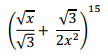Solution:

[(√x/ √3) + (√3/ 2x2)]15

We know that the general term of (a + b)n is:

Tr + 1 = nCr (a)n – r (a)r

Tr + 1 = 15Cr (√x/ √3)15 – r . (√3/ 2x2)r

= 15Cr (x/3) (15 – r)/2 . (3)r/2 [1/ 2r x2r]

= 15Cr (3)[(r/2) – (15 – r)/2] 2-r x[(15 – r)/2 – 2r]

To get the term which is independent of x, assume the value of power of x as 0.

[(15 – r)/2 – 2r] = 0

15 – r – 4r = 0

5r = 15

r = 3

Thus, the third term will be the required term.

T3 + 1 = 15C3 [3(3/2) – 6]/ (2)3

= 15C3 [3(-9/2)]/ 8

= 455 × (1/ 39/2 × 8)

Question 8

Find the equation of acute angled bisector of lines:

3x – 4y + 7 = 0 and 12x – 5y – 8 = 0

Solution:

Given,

3x – 4y + 7 = 0

12x – 5y – 8 = 0

Here,

a1 = 3, b1 = -4, c1 = 7

a2 = 12, b2 = -5, c2 = -8

a1a2 + b1b2 = 3(12) + (-4)(-5)

= 36 + 20

= 56 > 0

For acute angle, take a positive sign

(a1x + b1y + c1)/ √(a12 + b12) = -(a2x + b2y + c2)/ √(a22 + b22)

(3x – 4y + 7)/ √(9 + 16) = -(12x – 5y – 8)/√(144 + 25)

(3x – 4y + 7)√25 = -(12x – 5y – 8)/ √169

(3x – 4y + 7)/5 = -(12x – 5y – 8)/13

13(3x – 4y + 7) = -5(12x – 5y – 8)

39x – 52y + 91 = -60x + 25y + 40

39x – 52y + 91 + 60x – 25y – 40 = 0

99x – 77y + 51 = 0

Question 9

(a) Find the equation of the tangent to the circle

x2 + y2 – 2x – 2y – 23 = 0 and parallel to line 2x + y + 3 = 0

Solution:

x2 + y2 – 2x – 2y – 23 = 0 and parallel to line 2x + y + 3 = 0

Given,

x2 + y2 – 2x – 2y – 23 = 0

Centre = (1, 1)

Radius = √[(1)2 + (1)2 + 23] = √(1 + 1 + 23) = √25 = 5

Let 2x + y + k = 0 be the equation of tangent parallel to 2x + y + 3 = 0 (given).

2x + y + k = 0 is a tangent to the circle and the perpendicular distance from the centre of the circle (1, 1) to this line is equal to the radius 5.

⇒ |2(1) + 1 + k|/ √(22 + 12) = 5

|2 + 1 + k|/ √(4 + 1) = 5

|3 + k| = 5√5

3 + k = 5√5 or 3 + k = -5√5

k = -3 + 5√5 or k = -(k + 5√5)

Hence, the equations of tangents are 2x + y – 3 + 5√5 = 0 and 2x + y – (3 + 5√5) = 0.

OR

(b) Find the equation of the circle which passes through the points (2, 3), (4, 5), and the centre lies on the line y – 4x + 3 = 0.

Solution:

Let x² + y² + 2gx + 2fy + c = 0 be the equation of the circle where (-g, -f) is the centre of the circle.

Given,

The circle passes through the points (2, 3) and (4, 5).

2² + 3² + 2g(2) + 2f(3) + c = 0

4g + 6f + c + 13 = 0 …….(i)

And

4² + 5² + 2g(4) + 2f(5) + c = 0

8g + 10f + c + 41 = 0 ………(ii)

Solving (i) and (ii),

4g + 4f + 28 = 0

⇒ g + f + 7 = 0 ……….(iii)

Also, given that centre of circle lies on the line y – 4x + 3 = 0.

-f +4g + 3 = 0…….(iv)

Solving (iii) and (iv),

5g + 10 = 0

g = -2

⇒ f = -7 – g = – 7 + 2 = -5

Substituting f = -5 and g = -2 in (ii),

8(-2) + 10(-5) + c + 41 = 0

-16 – 50 + c + 41 = 0

c = 25

Therefore, the equation of the circle is:

x2 + y2 + 2(-2)x + 2(-5)y + 25 = 0

x2 + y2 – 4x – 10y + 25 = 0

Question 10

Differentiate the function sin (2x – 3) by the First Principle of differentiation.

Solution:

Let f(x) = sin (2x – 3)

And

f(x + h) = sin [2(x + h) – 3]

By the First Principle of differentiation,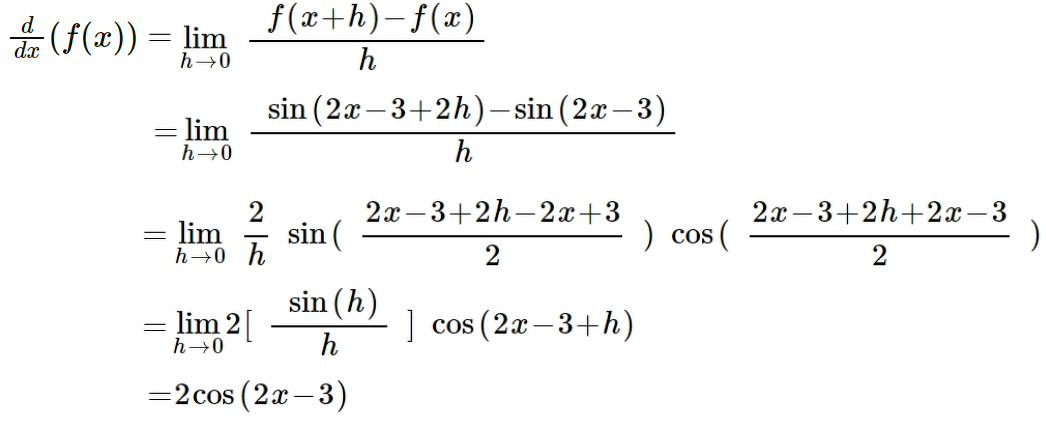Question 11

In a ΔABC, (b2 – c2)/ (b2 + c2) = sin (B – C)/ sin (B + C), prove that it is either a right angled triangle or isosceles Δ.

Solution:

In a triangle ABC,

a/sin A = b/sin B = c/sin C

Let a/sin A = b/sin B = c/sin C = k

⇒ a = k sin A, b = k sin B, c = k sin C

Given,

(b2 – c2)/ (b2 + c2) = sin (B – C)/ sin (B + C)

Using componendo and dividendo rule,

(b2 – c2 + b2 + c2)/ (b2 – c2 – b2 – c2) = [sin (B – C) + sin (B + C)] / [sin (B – C) – sin (B + C)]

2b2/ (-2c2) = (2 sin B cos C)/ (-2 cos B sin C)

b2/c2 = (sin B cos C)/ (cos B sin C)

(k2 sin2B)/ (k2 sin2C) = (sin B cos C)/ (cos B sin C)

sin B/ sin C = cos C/ cos B

sin B cos B = sin C cos C

Multiplying by 2 on both sides,

2 sin B cos B = 2 sin C cos C

sin 2B = sin 2C

sin 2B – sin 2C = 0

2 cos (B + C) sin (B – C) = 0

cos (B + C) = 0 or sin (B – C) = 0

cos (B + C) = cos 90°

B + C = 90°….(i)

We know that,

A + B + C = 180°

A + 90° = 180°

A = 90°

And

sin (B – C) = sin 0

B – C = 0°

B = C….(ii)

From (i) and (ii),

B = C = 45°

Hence, ΔABC is an isosceles right angled triangle.

Question 12

(a) If ‘x’ be real, find the maximum and minimum value of y = (x + 2)/ (2x2 + 3x + 6).

Solution:

Let y = f(x) = (x + 2)/ (2x2 + 3x + 6)

f'(x) = [(2x2 + 3x + 6)(1) – (x + 2)(4x + 3)]/ (2x2 + 3x + 6)2

Let f'(x) = 0

2x2 + 3x + 6 – 4x2 – 3x – 8x – 6 = 0

-2x2 – 8x = 0

2x2 + 8x = 0

2x(x + 4) = 0

x = 0, x = -4

f”(x) = d/dx [(-2x2 – 8x)/ (2x2 + 3x + 6)2]

= [(2x2 + 3x + 6)2 (-4x – 8) – (-2x2 – 8x) (2)(2x2 + 3x + 6)(4x + 3)]/ (2x2 + 3x + 6)4

At x = 0, f”(x) = -(62 × 8)/ 64 < 0 Maxima

x = -f f”(x) > 0 Minima

At x = 0 we will get the greatest value and at x = -4 we will get the least value.

f(x) = (x + 2)/ (2x2 + 3x + 6)

Maximum value of f(x) = (0 + 2)/ [2(0)2 + 3(0) + 6] = 2/6 = 1/3

Minimum value of f(x) = (-4 + 2)/ [2(-4)2 + 3(-4) + 6]

= -2/ (32 – 12 + 6)

= -2/26

= -1/13

OR

(b) If α, β be the roots of x2 + lx + m = 0, then form an equation whose roots are (α + β)2 and (α – β)2.

Solution:

Given,

α, β are the roots of x2 + lx + m = 0.

Sum of roots = α + β = -l/1 = -l

Product of roots = αβ = m/1 = m

Now,

(α + β)2 = (-l)2 = l2

(α – β)2 = (α + β)2 – 4αβ = l2 – 4m

(α + β)2 + (α – β)2 = l2 + l2 – 4m = 2l2 – 4m

(α + β)2 (α – β)2 = l2(l2 – 4m) = l4 – 4l2m

The quadratic equation with roots (α + β)2 and (α – β)2 is:

x2 – (sum of the roots)x + (product of the roots) = 0

x2 – (2l2 – 4m)x + l4 – 4l2m = 0

Question 13

(a) The sum of three consecutive numbers of a G.P is 56. If we subtract 1, 7 and 21 from these numbers in the order, the resulting numbers form an A.P., find the numbers.

Solution:

Let a , ar , ar² be the three numbers in GP.

Given,

a + ar + ar² = 56

a(1 + r + r²) = 56….(i)

Also,

(a – 1) , (ar – 7), ( ar² – 21) are in AP.

Thus, the common difference is always constant.

(ar² – 21) – (ar – 7) = (ar – 7) – (a – 1)

ar² – ar – 21 + 7 = ar – a – 7 + 1

ar² – 2ar + a – 8 = 0

a( r² – 2r + 1) = 8….(ii)

Dividing (i) by (ii),

a( 1 + r + r²)/ [a(r² – 2r + 1)] = 56/8

(1 + r + r²)/(1 – 2r + r²) = 7

1 + r + r² = 7 – 14r + 7r²

6r² – 15r + 6 = 0

2r² – 5r + 2 = 0

2r² – 4r – r + 2 = 0

2r( r – 2) -(r – 2) = 0

(2r – 1)(r – 2) = 0

r = 2, 1/2

If r = 2 then, equ (i) becomes,

a(1 + 2 + 4) = 56

7a = 56

a = 8

Therefore, numbers are:

a = 8

ar = 8 × 2 = 16

ar² = 8 × 2² = 32

Similarly, if r = ½,

a[1 + (½) + (¼)] = 56

7a/4 = 56

a = 8 × 4

a = 32

Therefore, the numbers are:

a = 32

ar = 32 × (½) = 16

ar² = 32 × (1/2²) = 32/4 = 8

Hence, the numbers are 32 , 16 , 8 or 8, 16, 32.

OR

(b) Find the sum of the series to n terms: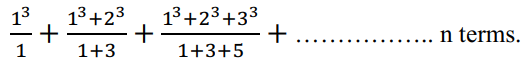Solution:

1³/1 + (1³ + 2³)/(1 + 3) + ( 1³ + 2³ + 3³)/(1 + 3 + 5)+…..

Tₙ = (nth term of the numerator) /(nth term of the denominator)

= (1³ + 2³ + 3³ + …… n terms) / (1 + 3 + 5 + …. n terms)

nth term of numerator = 1³ + 2³ + 3³ + ….. n terms

= ∑n³ = [n(n + 1)/2]²

nth term of denominator = 1 + 3 + 5 + …… + n terms

= n² (sum of first n odd numbers)

Tₙ = n²( n + 1)²/4n² = ( n + 1)²/4

Sn = ∑Tₙ

=1/4 ∑(n + 1)²

= 1/4 (∑n² + 2∑n + ∑1 )

Sₙ = 1/4 [{n(n + 1)(2n + 1)/6} +{2n(n + 1)/2} + n]

= 1/4 [{n(n + 1)(2n + 1)/6} + n(n + 1) + n]

= n/24 [2n2 + n + 2n + 1 + 6n + 6 + 6]

= n/24 [2n² + 9n + 13]

Hence, sum of the given series to n terms = n/24 [2n² + 9n + 13]

Question 14

Find the mean, standard derivation for the following data: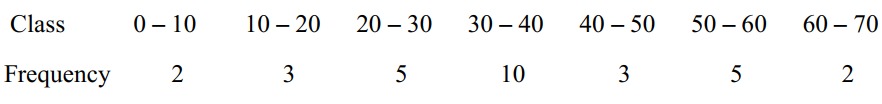Solution:

 Class Frequency (fi) Class mark (xi) fixi (xi – mean) = (xi – 35.67) (xi – mean)2 fi(xi – mean)2 0 – 10 2 5 10 -30.67 940.649 1881.298 10 – 20 3 15 45 -20.67 427.249 1281.747 20 – 30 5 25 125 -10.67 113.849 569.245 30 – 40 10 35 350 -0.67 0.4489 4.489 40 – 50 3 45 135 9.33 87.049 261.147 50 – 60 5 55 275 19.33 373.649 1868.245 60 – 70 2 65 130 29.33 860.249 1720.498 Total ∑fi = 30 ∑fixi = 1070 7856.669

Mean = ∑fixi/ ∑fi

= 1070/ 30

= 35.67

Variance = 1/N [∑fi(xi – mean)2]

= 1/30 (7856.669)

= 261.89

Standard deviation = √261.89 = 16.18

SECTION B

Question 15

(a) Find the coordinates of a point on the parabola y2 = 8x, whose focal distance is 4.

Solution:

Given,

Equation of parabola is y2 = 8x

Comparing with y2 = 4ax,

4a = 8

a = 2

Thus, the focus is S = (2, 0)

Let P(x, y) be any point on the parabola.

We know that,

Distance of any point on the parabola from the focus = Focal distance

√[(1 – 2)2 + (y – 0)2] = 4 (given)

√(x2 + 4 – 4x + y2) = 4

√(x2 + 4 – 4x + 8x) = 4 [since y2 = 8x]

√(x2 + 4x + 4) = 4

√(x + 2)2 = 4

x + 2 = ±4

x + 2 = 4, x1 + 2 = -4

x = 2, x1 = -6

x = -6 is not possible.

Therefore, x = 2

Now,

y2 = 8(2)

y2 = 16

y = ±4

Hence, the coordinates of the required points are (2, 4) and (2, -4).

(b) Prove that: ~(p ⇒ q) = p^(~q)

Solution:

 p q p ⇒ q ~(p ⇒ q) ~q p^(~q) T T T F F F T F F T T T F T T F F F F F T F T F

Therefore, ~(p ⇒ q) = p^(~q)

(c) Write Converse and inverse of the given conditional statement:

If a number n is even, then n2 is even.

Solution:

Given,

If a number n is even, then n2 is even.

Converse of the given conditional statement is:

If a number n2 is even, then n is even.

Inverse of the given conditional statement is:

If a number n is not even, then n2 is not even.

Question 16

(a) Find the equation of the ellipse whose focus (1, 2), directrix 3x + 4y – 5 = 0 and eccentricity is 1/2.

Solution:

Given,

Focus = S(1, 2)

Directrix is 3x + 4y – 5 = 0

Eccentricity (e) = 1/2

Let P(x, y) be any point on the ellipse.

We know that,

The perpendicular distance from the point (x1, y1) to the line ax + by + c = 0 = |ax1 + by1 + c|/ √(a2 + b2)

Here,

(x1, y1) = (x, y)

a = 3, b = 4, c = -5

And

SP = e (perpendicular distance)

SP2 = e2 (perpendicular distance)2

(x – 1)2 + (y – 2)2 = (1/2)2 [|3x + 4y – 5|/ √(32 + 42)]2

x2 – 2x + 1 + y2 – 4y + 4 = (1/4) [|3x + 4y – 5|2/ (9 + 16)]

x2 + y2 – 2x – 4y + 5 = (1/100) (9x2 + 16y2 + 25 + 24xy – 40y – 15x)

100x2 + 100y2 – 200x – 400y + 500 – 9x2 – 16y2 – 25 – 24xy + 40y + 15x = 0

91x2 + 84y2– 185x – 360y – 24xy + 475 = 0

Therefore, the equation of the ellipse is 91x2 + 84y2 – 24xy – 185x – 360y + 475 = 0.

OR

(b) Find the centre, focus, eccentricity and latus rectum of the hyperbola 16x2 – 9y2 = 144.

Solution:

Given,

Equation of hyperbola is:

16x2 – 9y2 = 144

(16x2/144) – (9y2/144) = 1

(x2/9) – (y2/16) = 1

This is of the form (x2/a2) – (y2/b2) = 1

Therefore, the centre is (0, 0).

Now,

a2 = 9, b2 = 16

b2 = a2(e2 – 1)

16 = 9(e2 – 1)

e2 – 1 = 16/9

e2 = (16/9) + 1

e2 = 25/9

e = 5/3

Coordinates of foci = (±ae, 0) = (±5, 0)

Eccentricity = e = 5/3

Latus rectum = 2b2/a = 2(16)/ 3

= 32/3

Question 17

(a) In what ratio the point P(-2, y, z) divides the line joining the points A(2, 4, 3) and B(-4, 5, -6). Also, find the coordinates of point P.

Solution:

Let P(-2, y, z) divide the line joining the points A(2, 4, 3) and B(-4, 5, -6) in the ratio m : n.

Here,

(x1, y1, z1) = (2, 4, 3)

(x2, y2, z2) = (-4, 5, -6)

Using section formula,

P(x, y, z) = [(mx2 + nx1)/ (m + n), (my2 + ny1)/ (m + n), (mz2 + nz1)/ (m + n)]

(-2, y, z) = [(-4m + 2n)/ (m + n), (5m + 4n)/ (m + n), (-6m + 3n)/ (m + n)]

By equating the corresponding coordinates,

-4m + 2n = -2(m + n)

-4m + 2n = -2m – 2n

2n + 2n = -2m + 4m

4n = 2m

⇒ 2m = 4n

⇒ m/n = 4/2

⇒ m : n = 2 : 1

And

y = (5m + 4n)/ (m + n)

= (10 + 4)/ (2 + 1)

= 14/3

z = (-6m + 3n)/ (m + n)

= (-12 + 3)/ (2 + 1)

= -9/3

= -3

Hence, the required ratio is 2 : 1 and the coordinates of P are (-2, 14/3, -3).

OR

(b) If the origin is the centroid of the triangle with vertices (-4, 2, 6) (2a, 3b, 2c) and (8, 14, -10) find the values of a, b and c.

Solution:

Let the given vertices of a triangle be:

(-4, 2, 6) = (x1, y1, z1)

(2a, 3b, 2c) = (x2, y2, z2)

(8, 14, -10) = (x3, y3, z3)

Centroid = [(x1 + x2 + x3)/3, (y1 + y2 + y3)/3, (z1 + z2 + z3)/3]

= [(-4 + 2a + 8)/3, (2 + 3b + 14)/3, (6 + 2c – 10)/3]

= [(4 + 2a)/3, (16 + 3b)/3, (-4 + 2c)/3]

According to the given,

[(4 + 2a)/3, (16 + 3b)/3, (-4 + 2c)/3] = (0, 0, 0)

By equating the corresponding coordinates,

(4 + 2a)/3 = 0

4 + 2a = 0

2a = -4

a = -4/2 = -2

Now,

(16 + 3b)/3 = 0

16 + 3b = 0

3b = -16

b = -16/3

And

(-4 + 2c)/3 = 0

-4 + 2c = 0

2c = 4

c = 4/2 = 2

Therefore, a = -2, b = -16/3, and c = 2.

Question 18

Find the equation of Parabola whose directrix is 2x – 3y + 4 = 0 and vertex at (5 – 4).

Solution:

Given,

Vertex (V) = (5, -4)

Directrix is 2x – 3y + 4 = 0

Let P(x, y) be any point on the parabola and F(x1, y1) be the focus.

Let us find the equation of the axis of parabola, which is perpendicular to the given directrix.

Thus, the equation which is perpendicular to the give directrix is 3x + 2y + λ = 0

This line passes through the vertex (5, -4).

3(5) + 2(-4) + λ = 0

15 – 8 + λ = 0

7 + λ = 0

λ = -7

Therefore, the equation of the axis of the parabola is 3x + 2y – 7 = 0.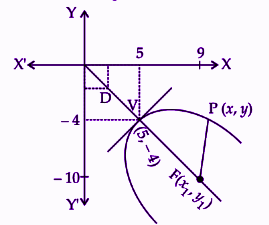The point of intersection of the given directrix and the axis of parabola is D= (1, 2).

Vertex = Midpoint of directrix and focus

(5, -4) = [(1 + x1)/2, (2 + y1)/2]

⇒ 1 + x1 = 10, 2 + y1 = -8

⇒ x1 = 9, y1 = -10

⇒ F(x1, y1) = (9, -10)

We know that,

PF2 = PM2

(x – 9)2 + (y + 10)2 = [|2x – 3y + 4|/√{22 + (-3)2}]2

x2 + 81 – 18x + y2 + 100 + 20y = (4x2 + 9y2 + 14 – 12xy – 24y + 16x)/13

13x2 + 1053 – 234x + 13y2 + 1300 + 260y = 4x2 + 9y2 + 14 – 12xy – 24y + 16x

13x2 + 1053 – 234x + 13y2 + 1300 + 260y – 4x2 – 9y2 – 14 + 12xy + 24y – 16x

9x2 + 4y2 + 12xy – 250x + 284y + 2337 = 0

SECTION C

Question 19

(a) The mean weight of 150 students in a certain class is 60 kg. The mean weight of boys is 70 kg and that of girls in the class is 55 kg. Find the number of boys and girls in the class.

Solution:

Given,

Mean weight of 150 students = 60 kg

Mean weight of boys = 70 kg

Mean weight of girls = 55 kg

Let x and y be the number of boys and girls in the class respectively.

Thus, x + y = 150

x = 150 – y….(i)

According to the given,

60 = (70x + 55y)/ 150

70x + 55y = 60 × 150

70(150 – y) + 55y = 9000 [From (i)]

10500 – 70y + 55y = 9000

10500 – 9000 = 15y

15y = 1500

y = 1500/ 15

y = 100

Substituting y = 100 in (i),

x = 150 – 100 = 50

Hence, the number of boys are 50 and the number of girls are 100.

(b) Compute D3 and D7 for the following distribution:

 Marks 0 – 10 10 – 20 20 – 30 30 – 40 40 – 50 50 – 60 60 – 70 70 – 80 No. of students 3 10 17 7 6 4 2 1

Solution:

 Marks No. of students (frequency) Cumulative frequency 0 – 10 3 3 10 – 20 10 13 20 – 30 17 30 30 – 40 7 37 40 – 50 6 43 50 – 60 4 47 60 – 70 2 49 70 – 80 1 50

Here,

N = 50

3N/10 = (3 × 50)/10 = 15

Cumulative frequency greater than and nearest to 15 is 30 which lies in the interval 20 – 30.

l = 20

h = 10

f = 17

cf = 13

D3 = l + [{(3N/10) – cf}/ f] × h

= 20 + [(15 – 13)/ 17] × 10

= 20 + (20/17)

= 20 + 1.176

= 21.176

7N/10 = (7 × 50)/10 = 35

Cumulative frequency greater than and nearest to 35 is 37 which lies in the interval 30 – 40.

l = 30

h = 10

f = 7

cf = 30

D7 = l + [{(7N/10) – cf}/ f] × h

= 30 + [(35 – 30)/ 7] × 10

= 30 + (50/7)

= 30 + 7.14

= 37.14

OR

Calculate the mode from the following data:

 Marks 0 – 10 10 – 20 20 – 30 30 – 40 40 – 50 No. of students 5 15 30 8 2

Solution:

From the given data,

Maximum frequency = 30

Modal class is 20 – 30

Frequency of the modal class = f1 = 30

Frequency of the class preceding the modal class = f0 = 15

Frequency of the class succeeding the modal class = f2 = 8

Lower limit of the modal class = l = 20

Class height = h = 10

Mode = l + [(f1 – f0)/ (2f1 – f0 – f2)] × h

= 20 + [(30 – 15)/ (2 × 30 – 15 – 8)] × 10

= 20 + [15/ (60 – 23)] × 10

= 20 + (150/ 37)

= 20 + 4.054

= 24.054

Question 20

(a) In a sample of ‘n’ observations given that ∑d2 = 55 and rank correlation r = 2/3, then find the value of ‘n’.

Solution:

Given,

∑d2 = 55

Rank correlation = r = ⅔

We know that,

Rank correlation = 1 – [(6 × ∑d2)/ (n(n2 – 1)

2/3 = 1 – [(6 × 55)/ (n(n2 – 1)]

⇒ 330/ n(n2 – 1) = 1 – (2/3)

⇒ 330/ n(n2 – 1) = 1/3

⇒ n(n2 – 1) = 330 × 3

⇒ n(n2 – 1) = 990

⇒ n(n2 – 1) = 10 × 99

⇒ n(n2 – 1) = 10 × (100 – 1)

⇒ n(n2 – 1) = 10 × [(10)2 – 1]

⇒ n = 10

(b) Find the correlation coefficient r(x, y) if:

n = 10, ∑x = 60, ∑y = 60, ∑x2 = 400, ∑y2 = 580, ∑xy = 305

Solution:

Given,

n = 10

∑x = 60

∑y = 60

∑x2 = 400

∑y2 = 580

∑xy = 305

Correlation coefficient is: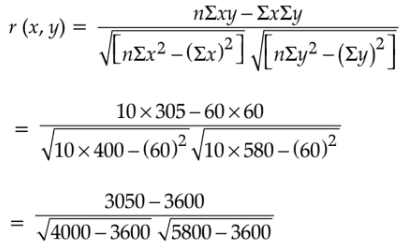= -550/ [√400 √2200]

= -550/ [20 × 10√22]

= -55/ (20 × 4.69)

= -55/93.8

= -0.586

OR

Ten students got the following percentages of marks in Mathematics and Physics:

 Mathematics 56 64 75 85 85 87 91 95 97 98 Physics 89 90 86 74 78 66 56 74 86 90

Find the Spearman’s rank correlation coefficient for the above data.

Solution:

 Mathematics Rank (R1) Physics Rank (R2) d = R1 – R2 d2 56 10 89 3 7 49 64 9 90 1.5 7.5 56.25 75 8 86 4.5 3.5 12.25 85 6.5 74 7.5 -1 1 85 6.5 78 6 0.5 0.25 87 5 66 9 -4 16 91 4 56 10 -6 36 95 3 74 7.5 -4.5 20.25 97 2 86 4.5 -2.5 6.25 98 1 90 1.5 -0.5 0.25

n = 10

∑d2 = 197.5

The spearman’s rank correlation coefficient = 1 – [(6 × ∑d2)/ n(n2 – 1)]

= 1 – [(6 × 197.5)/ 10(100 – 1)]

= 1 – [1185/ 10 × 99]

= 1 – (1185/990)

= (990 – 1185)/ 990

= -195/990

= -0.197

Question 21

Calculate the index number for the year 1990 with respect to 1980 as base from the following data using weighted average of price relatives:

 Commodity Weights Year 1990 Year 1980 A 22 320 200 B 48 120 100 C 17 20 28 D 13 60 40

Solution:

 Commodity Weights (w) Year 1990 (p1) Year 1980 (p0) Price relative I = (p1/p0) × 100 Iw A 22 320 200 160 3520 B 48 120 100 120 5760 C 17 20 28 71.43 1214.31 D 13 60 40 150 1950 Total ∑w = 100 ∑Iw = 12444.31

Index number of weighted average of price relatives = ∑Iw/ ∑w

= 12444.31/100

= 124.44

Question 22

The number of letters, in hundreds, posted in a certain city on each day for a week is given below:

 Mon. Tue. Wed. Thur. Fri. Sat. Sun. 35 70 36 59 62 60 71

Calculate 3 day moving averages and represent these graphically.

Solution:

 Day Number of letters (in 00’s) 3 days moving average Mon 35 Tue 70 (35 + 70 + 36)/3 = 141/3 = 47 Wed 36 (70 + 36 + 59)/3 = 165/3 = 55 Thur 59 (36 + 59 + 62)/3 = 157/3 = 52.33 Fri 62 (59 + 62 + 60)/3 = 181/3 = 60.33 Sat 60 (62 + 60 + 71)/3 = 193/3 = 64.33 Sun 71

Graph: